# Tamil Nadu SSLC Board (TN SSLC) Question Paper for Class 10th Maths 2019 In PDF

Tamil Nadu SSLC (Class 10) Maths 2019 question paper with solutions are given here in a pdf format at BYJU’S which can be downloaded so that students can easily access them. Students can get the TN SSLC Class 10 previous year question papers to practice as a part of the examination preparation. They are advised to solve the Tamil Nadu SSLC Board (TN SSLC) Question Paper for Class 10th Maths 2019 In PDF to get more acquainted with solving questions of different difficulty levels.

Students can also access model papers of Cass 10 Maths Tamilnadu board to be well versed with the new pattern of question paper. All these papers can be downloaded easily along with solutions. These solutions will help the students in verifying their answers after practicing 2019 Maths question paper for class 10.

### Tamil Nadu Board SSLC Class 10 Maths 2019 Question Paper with Solutions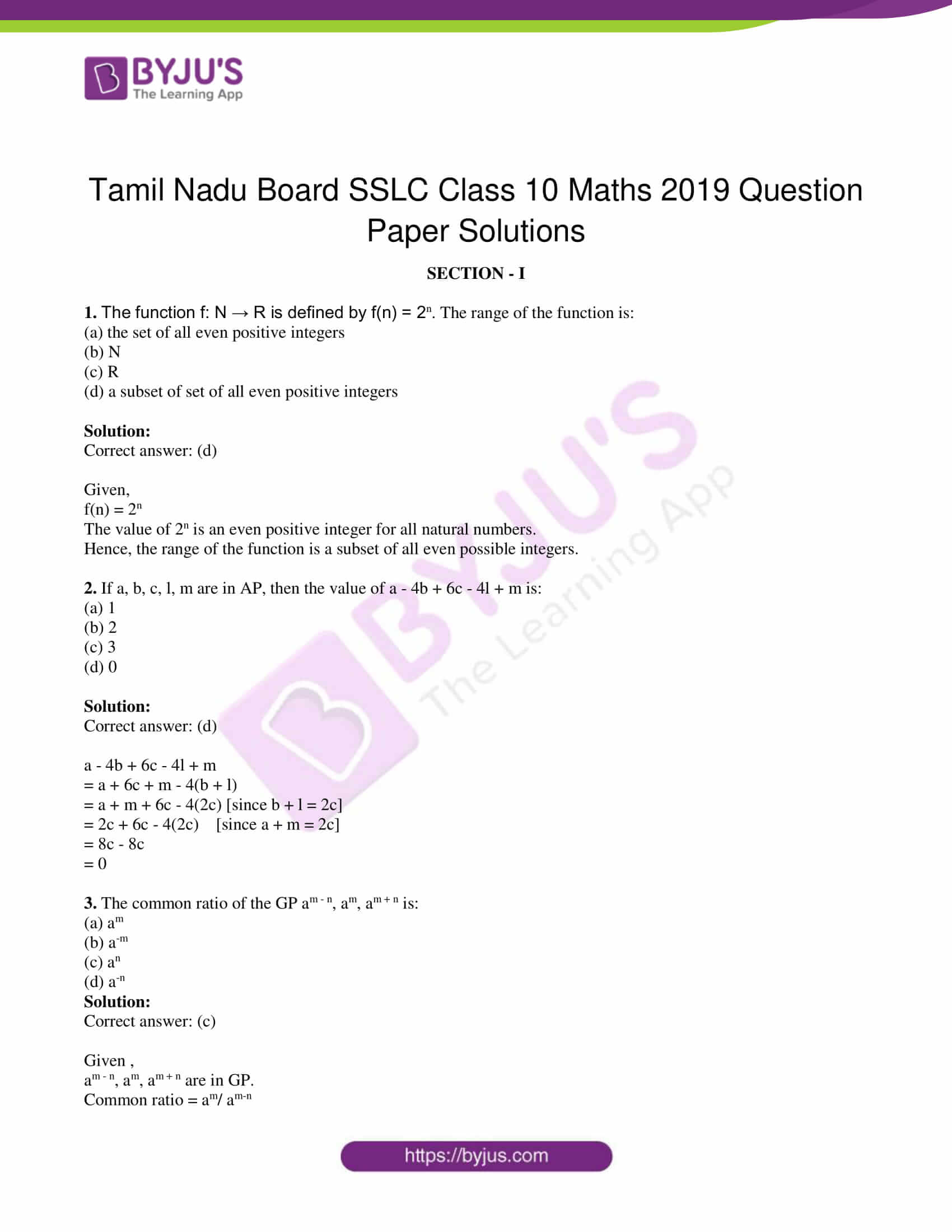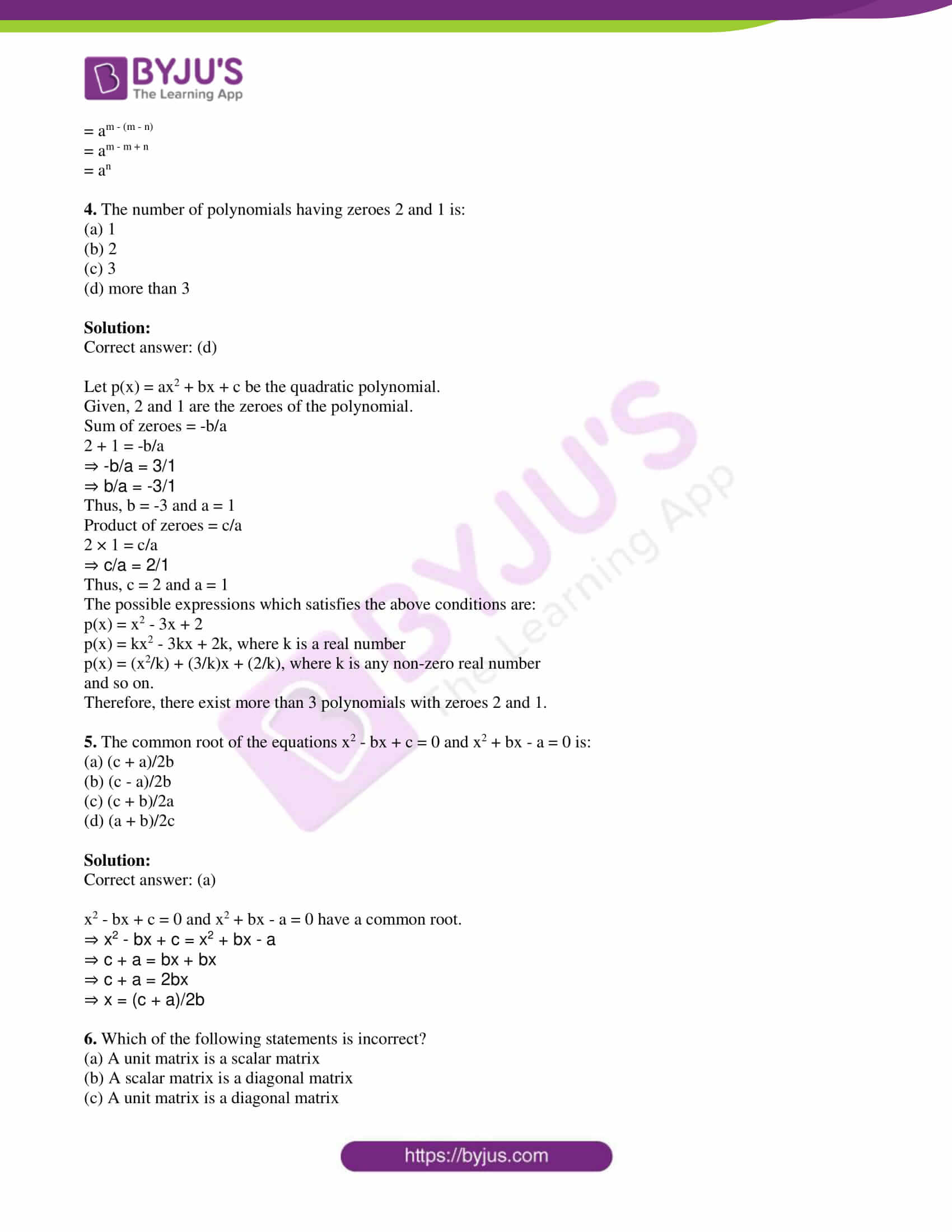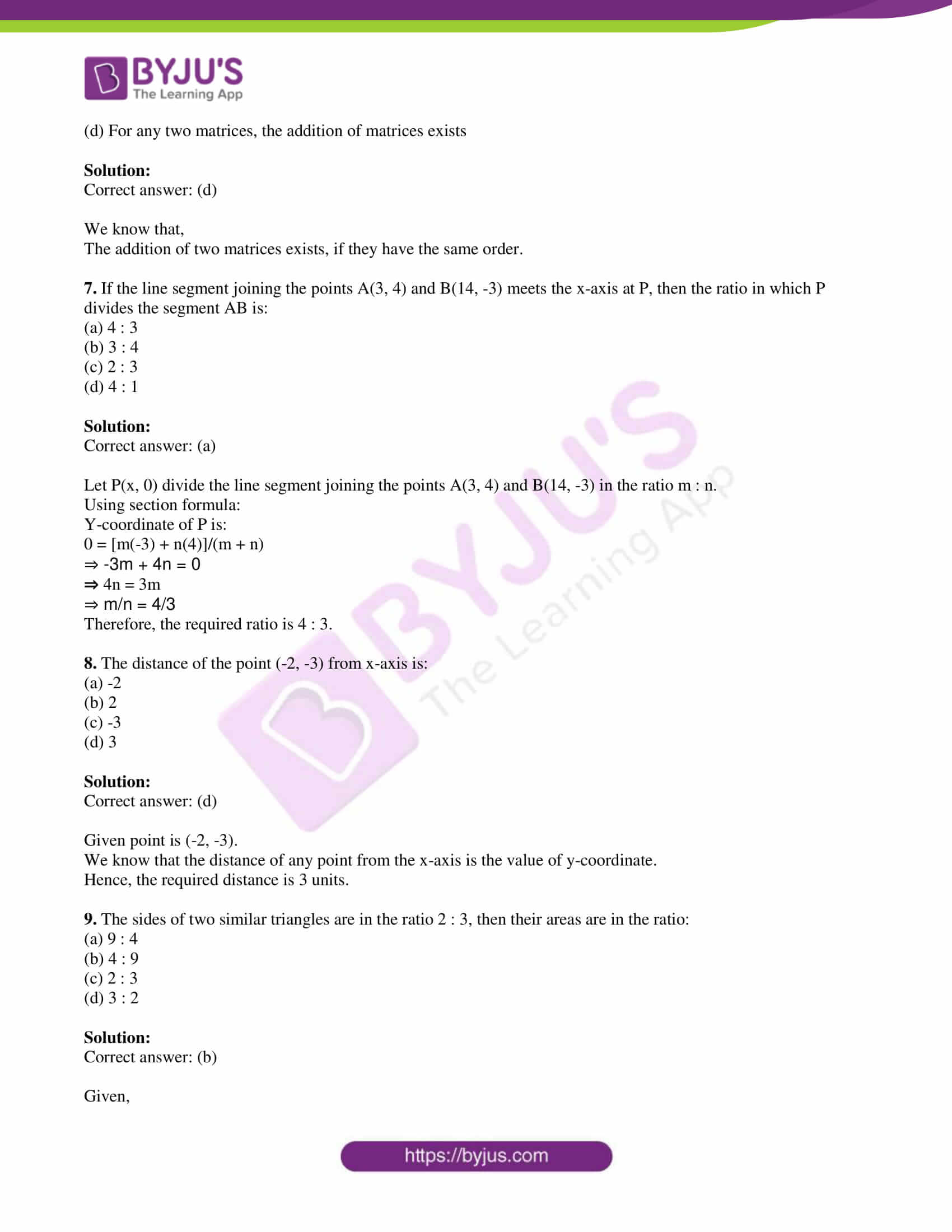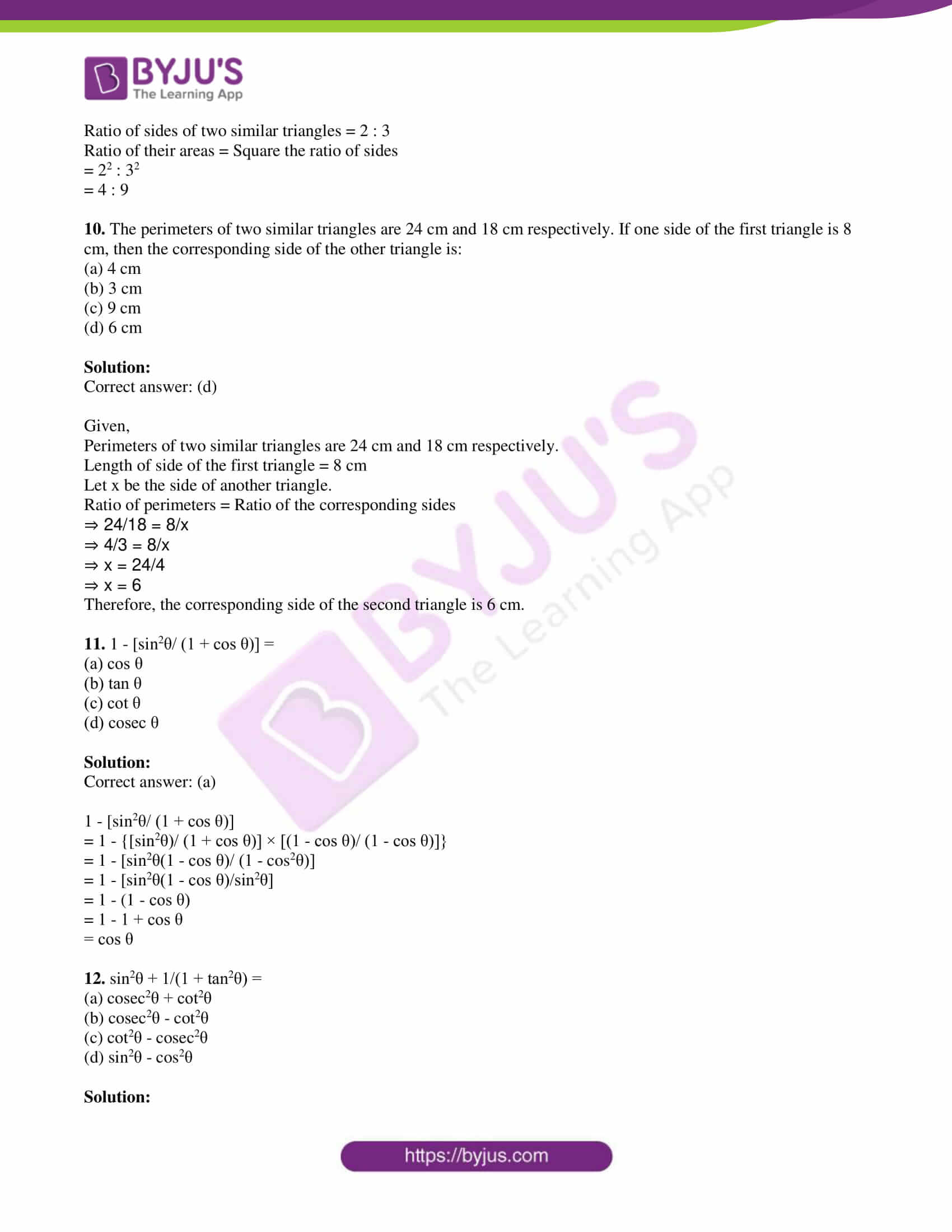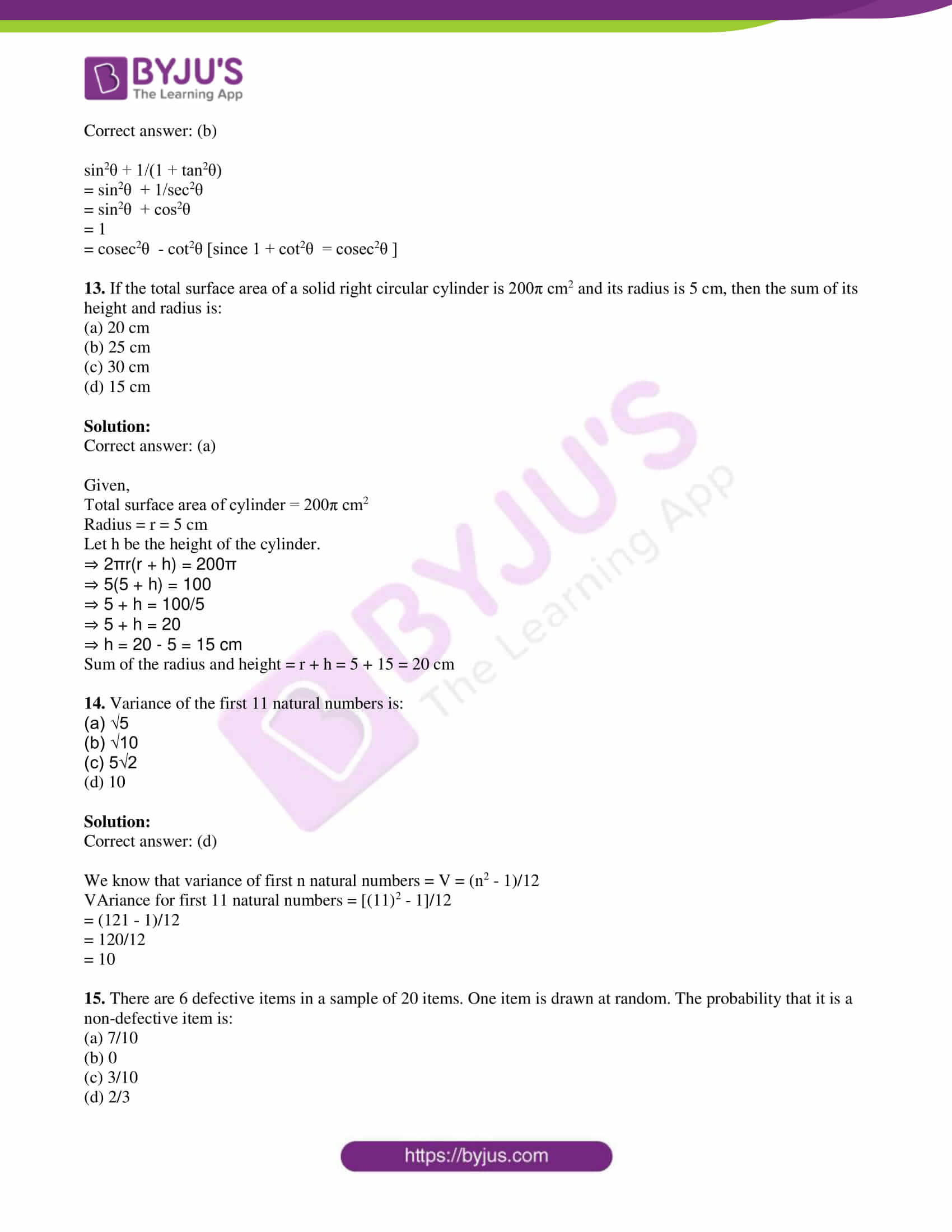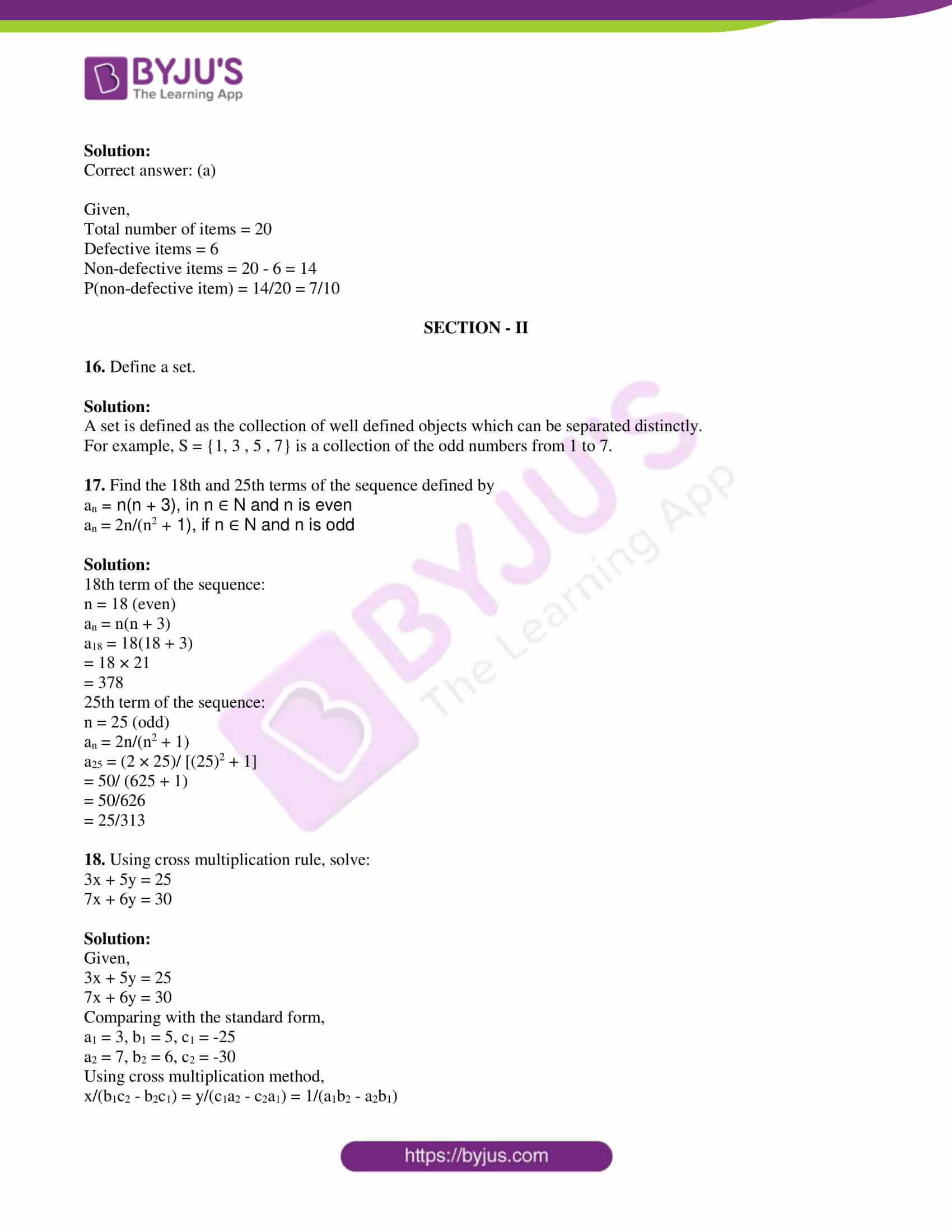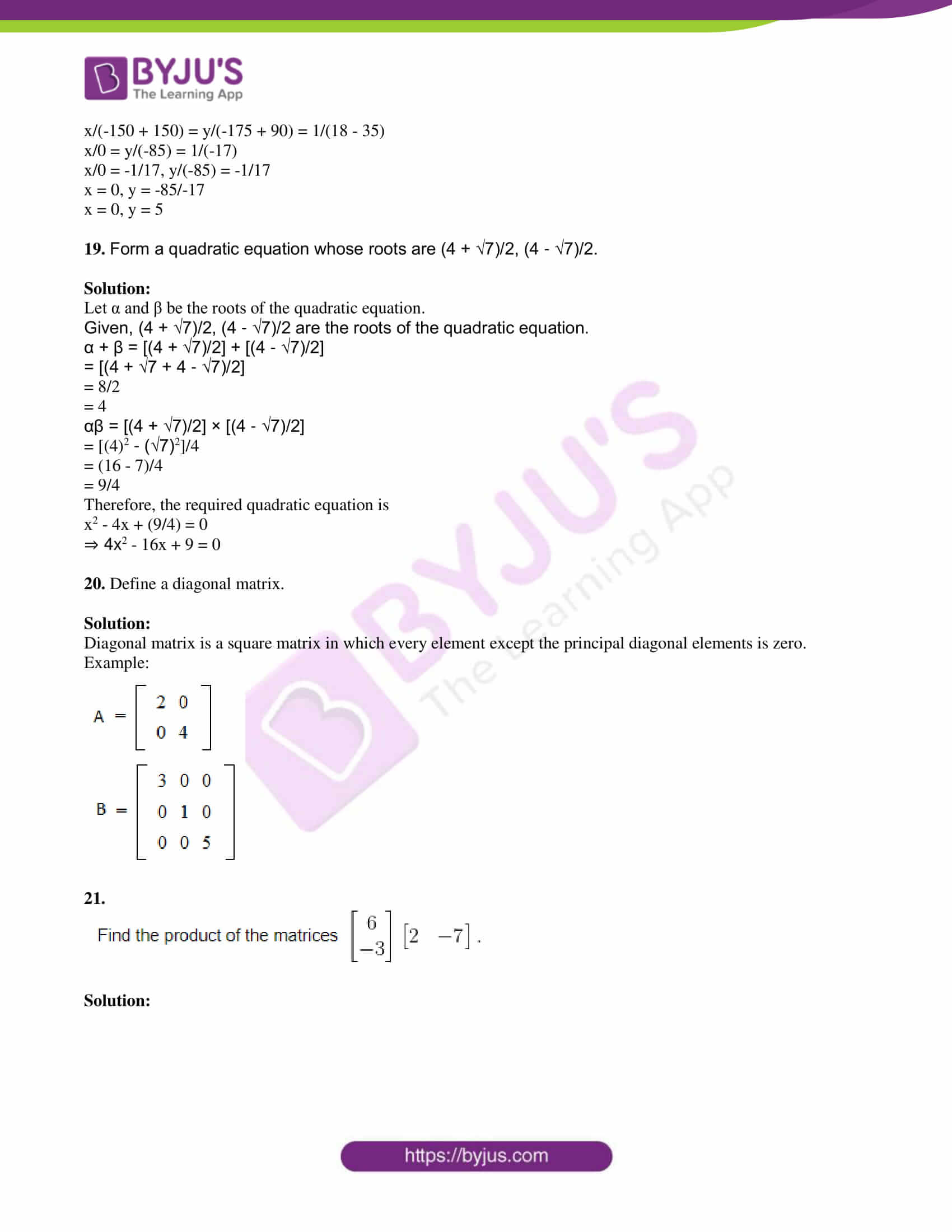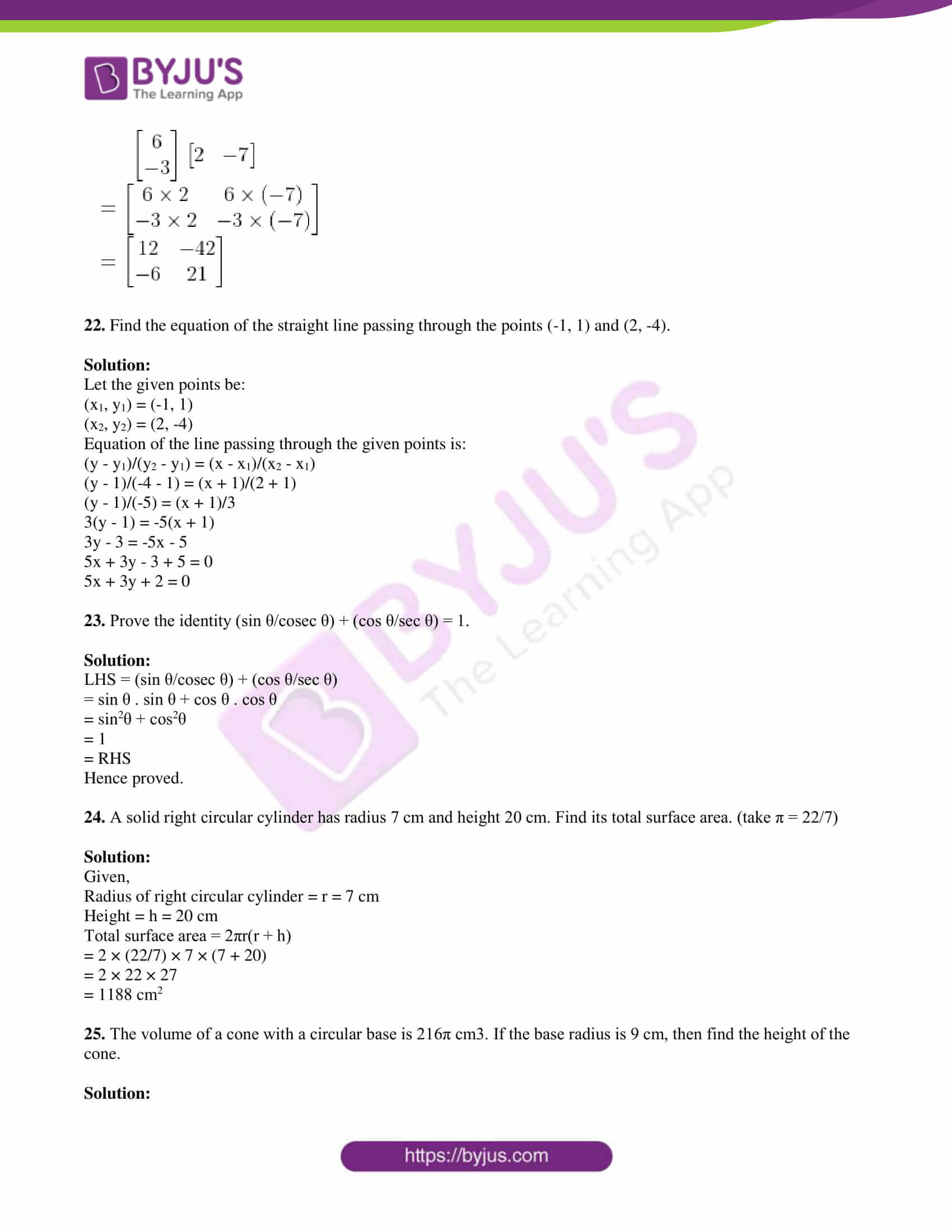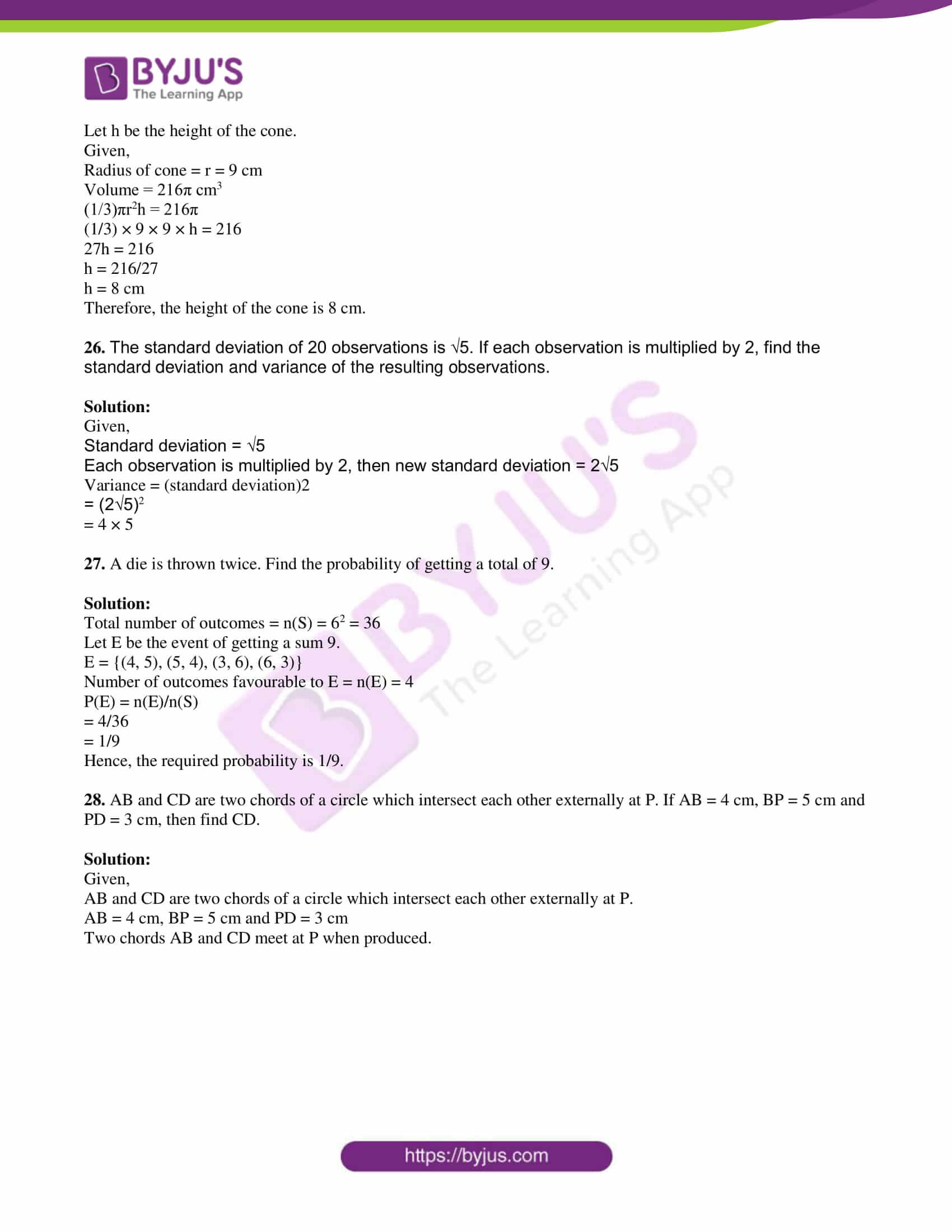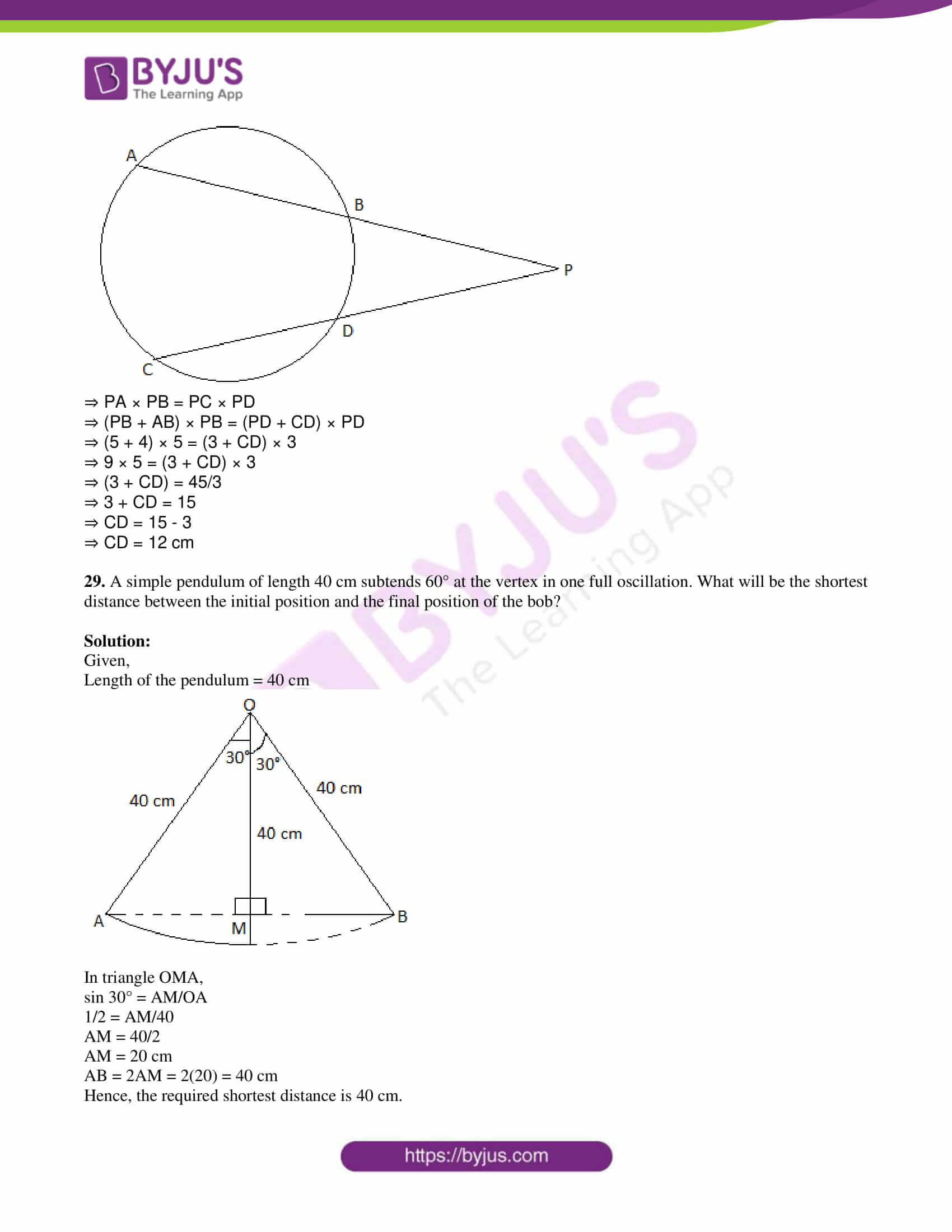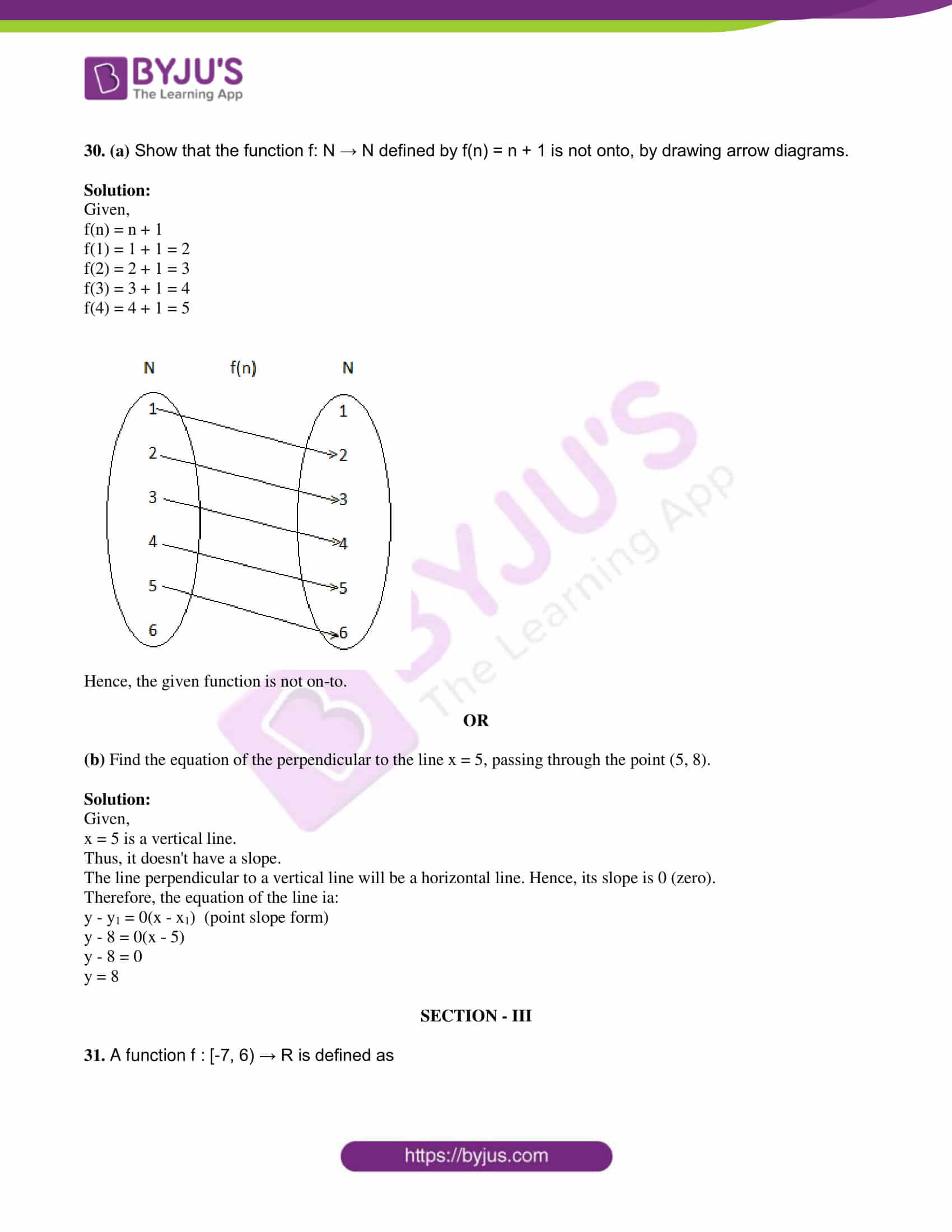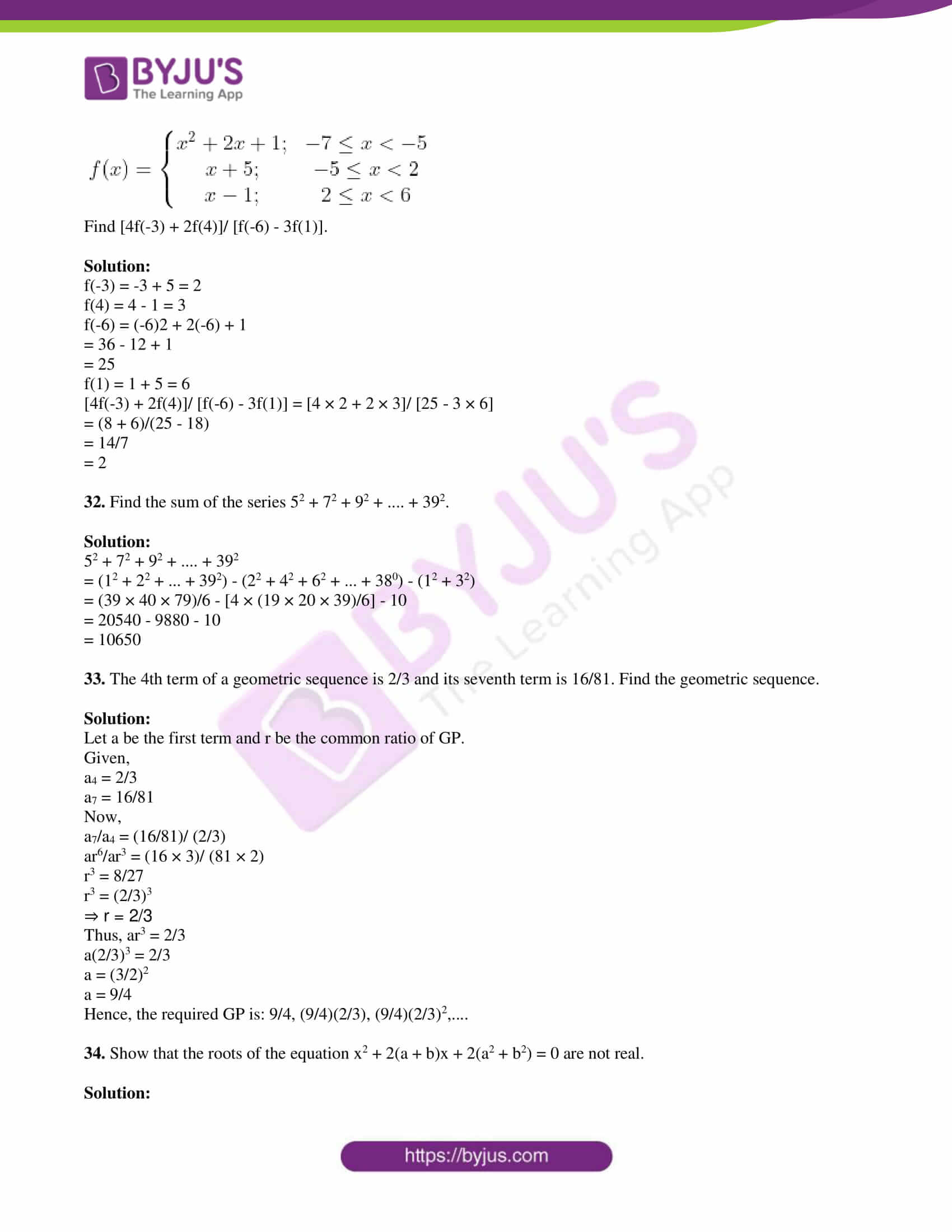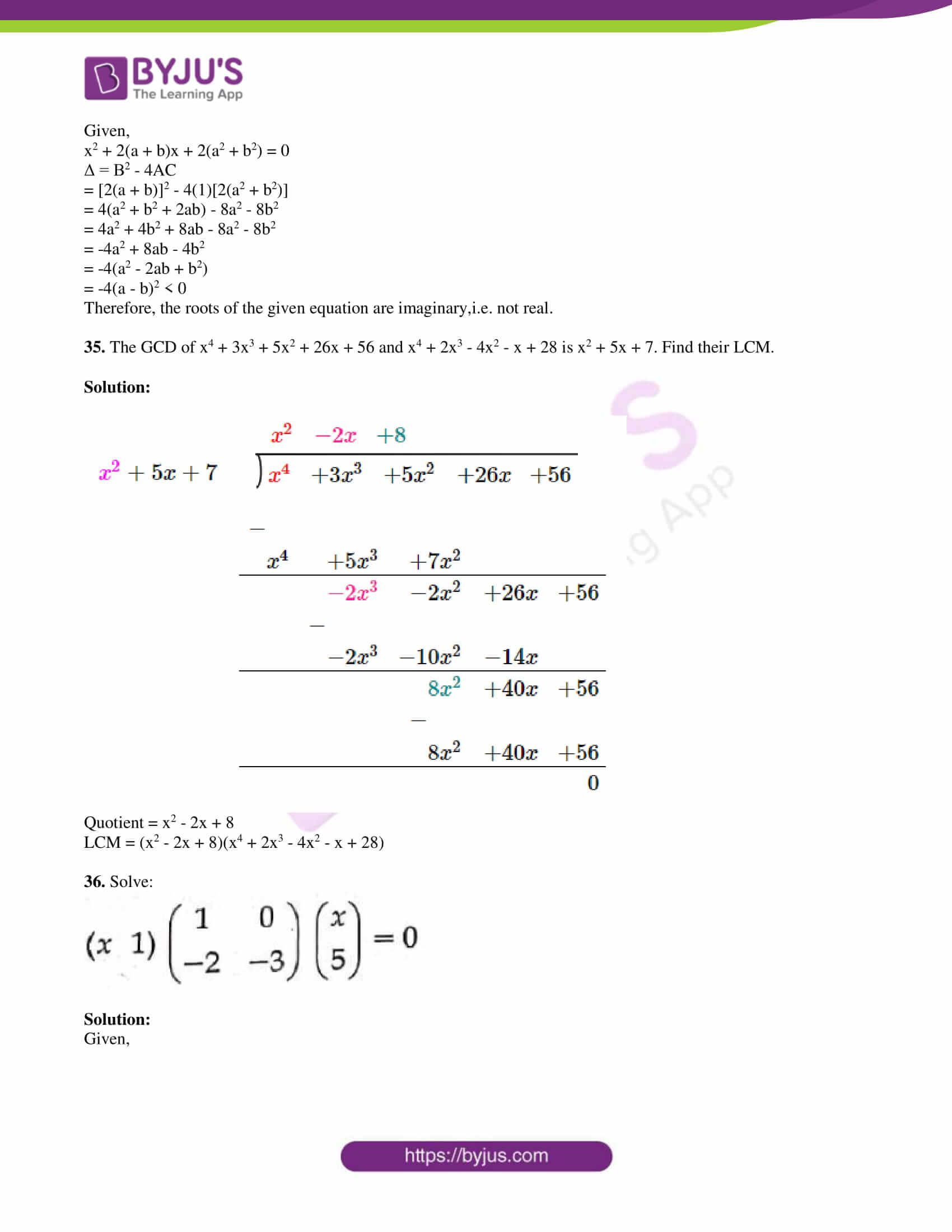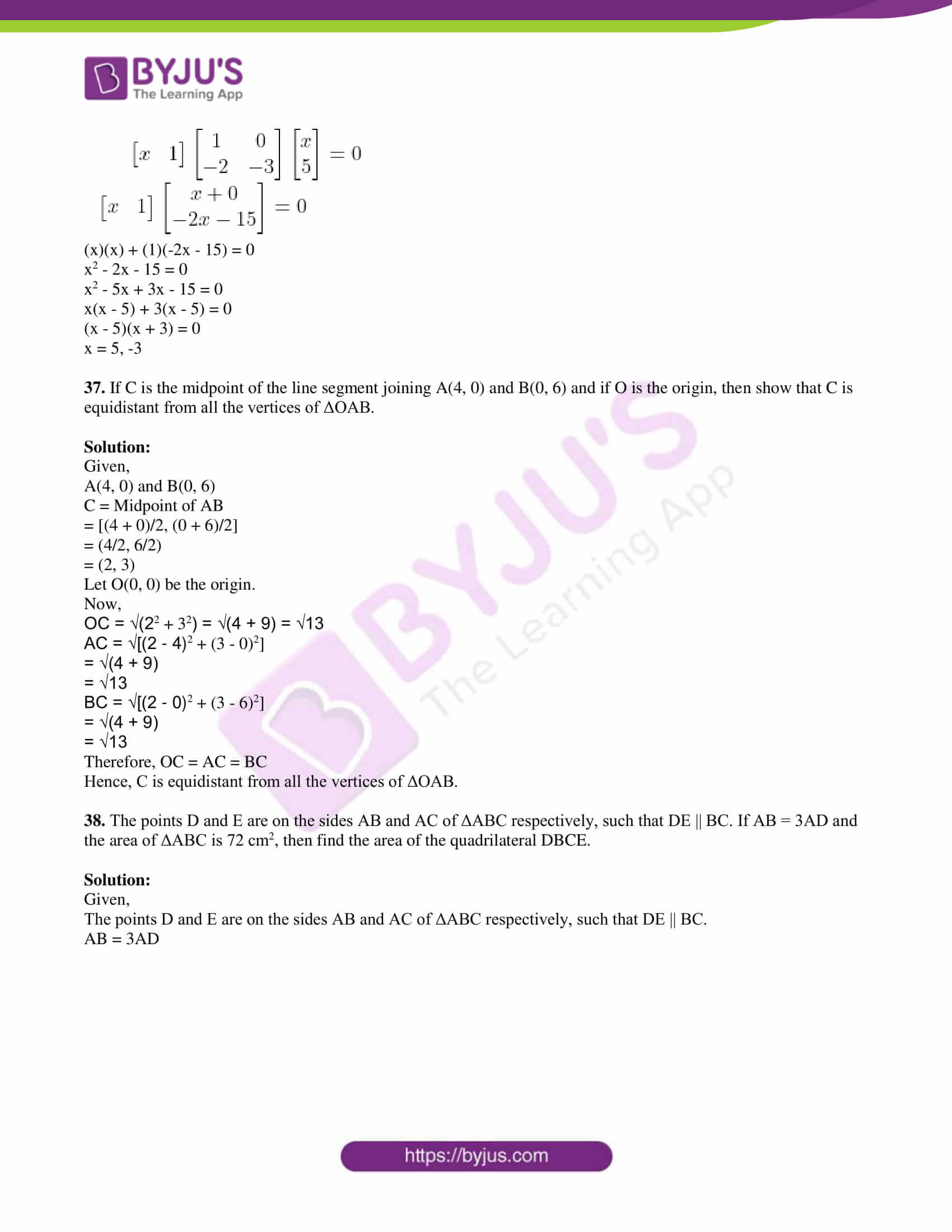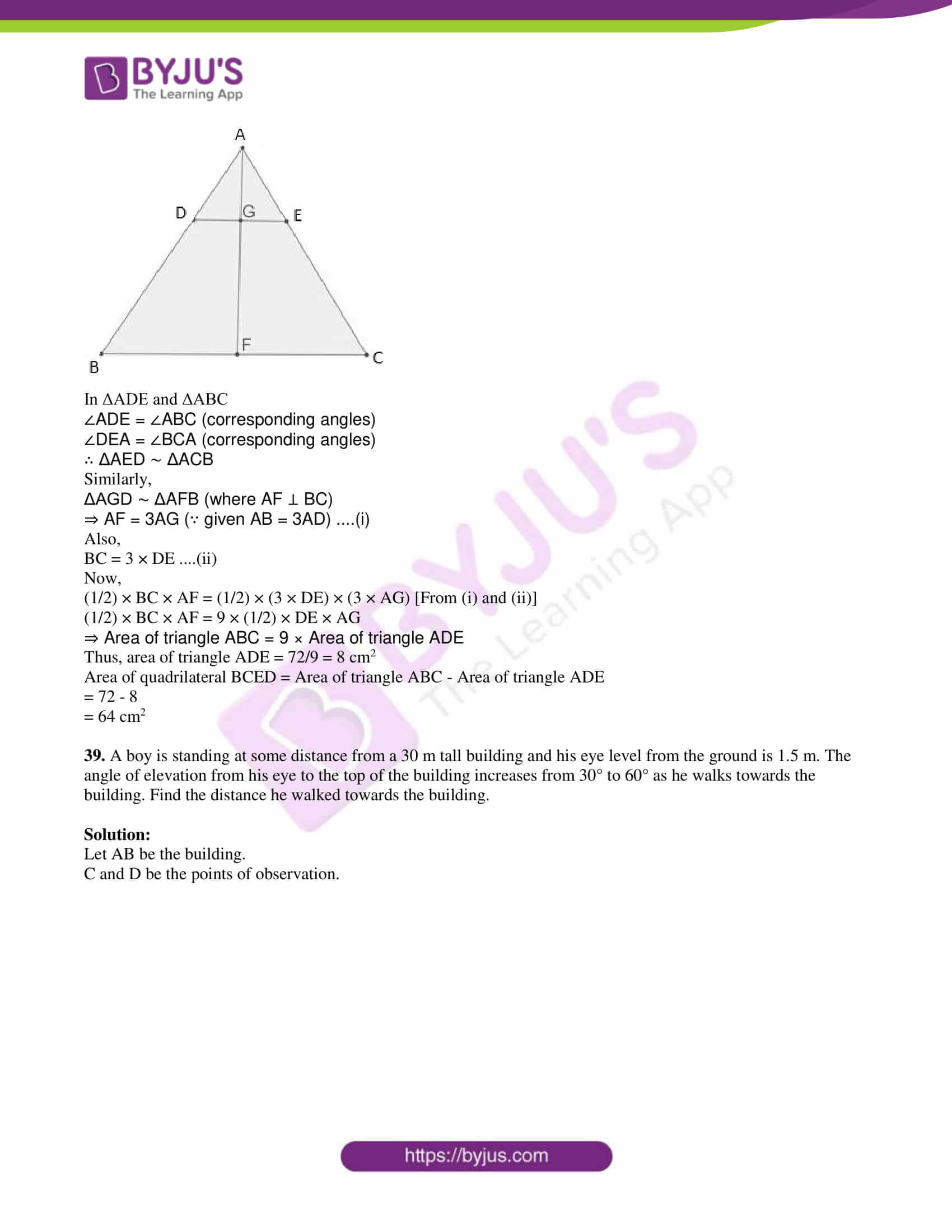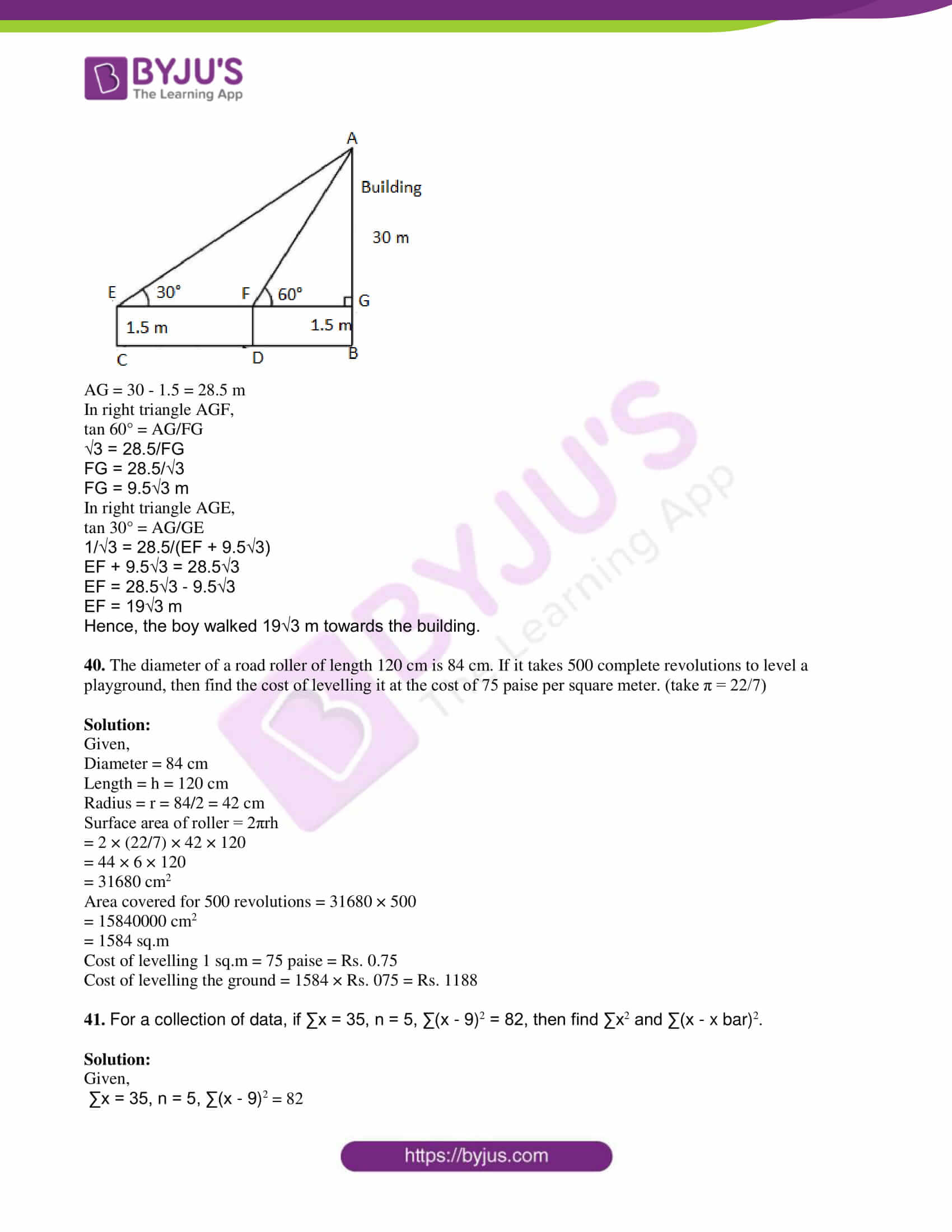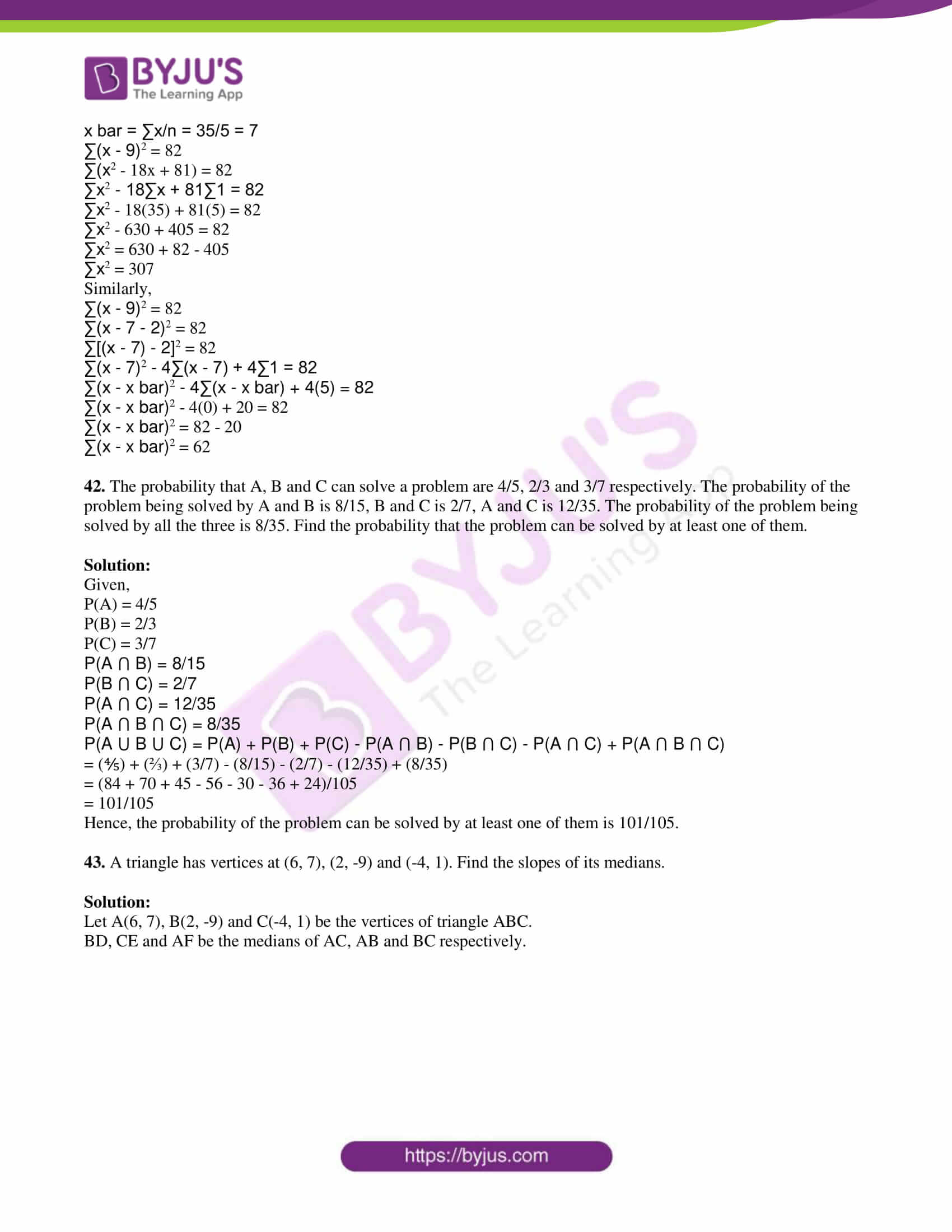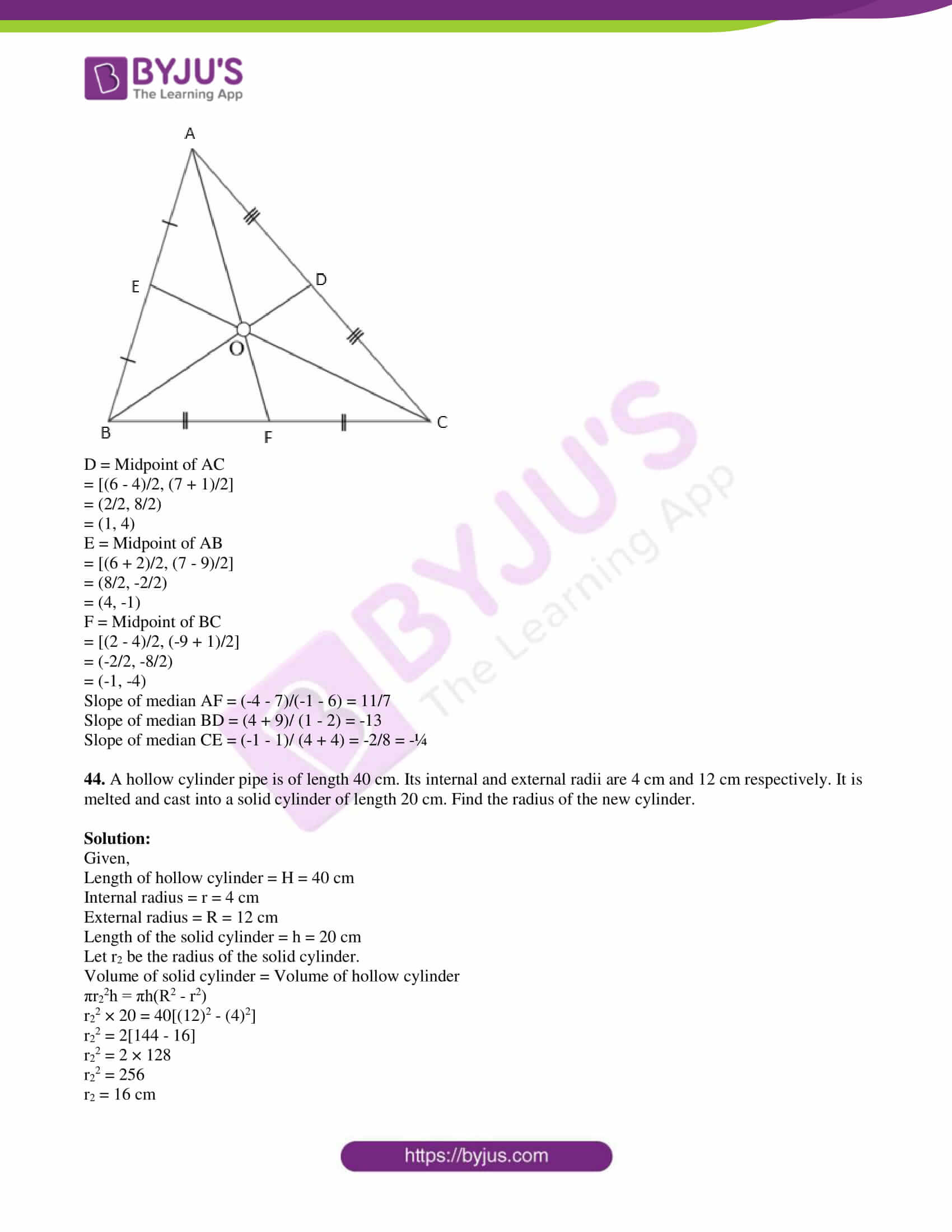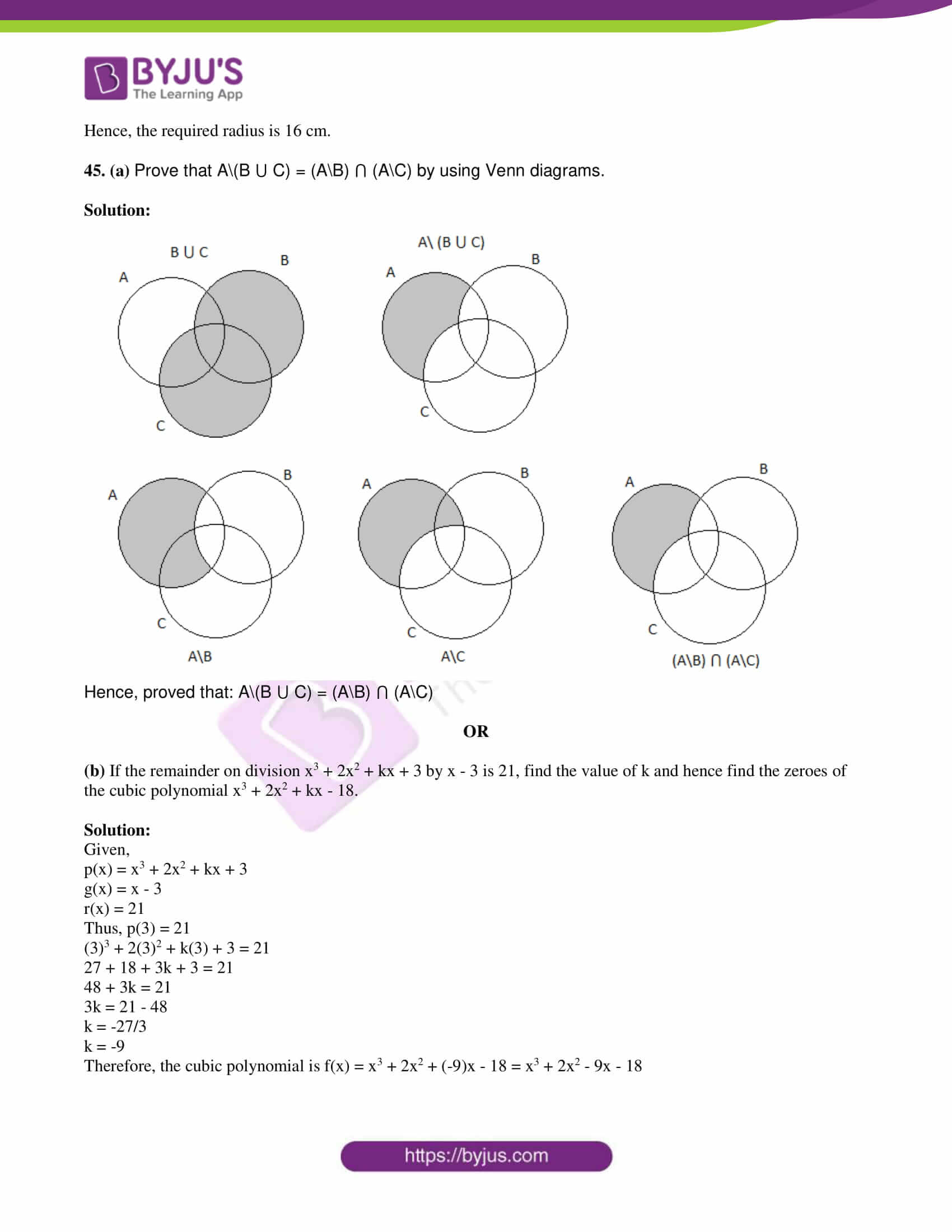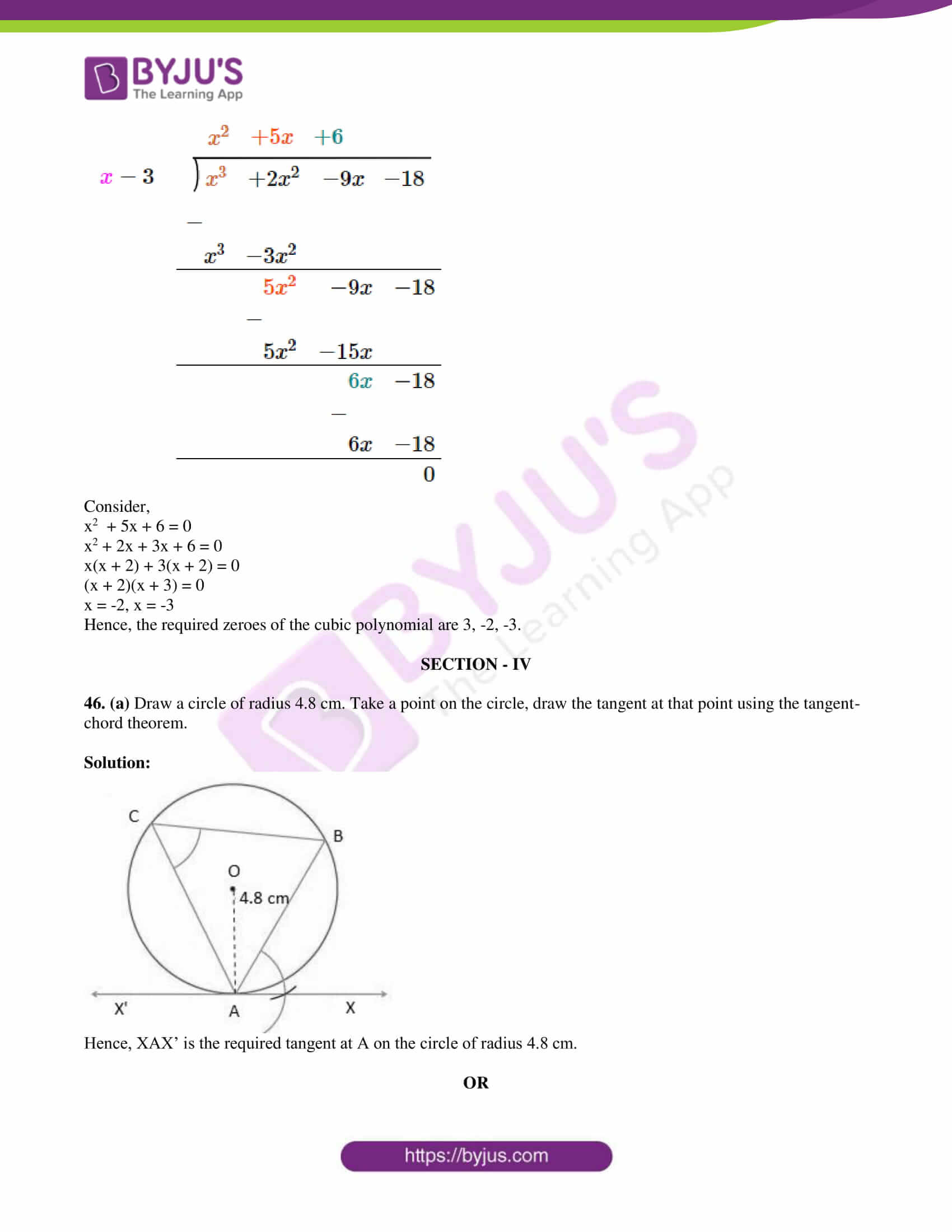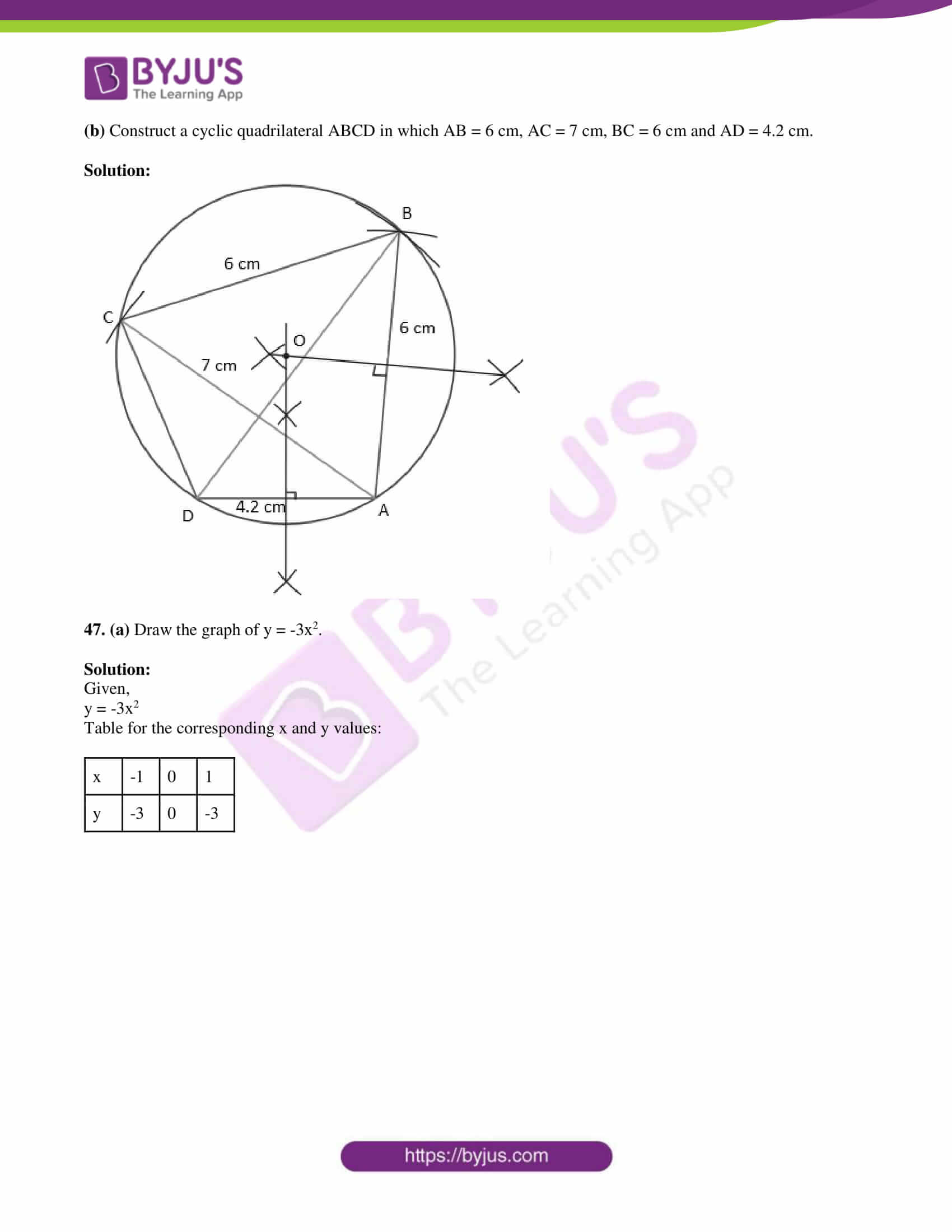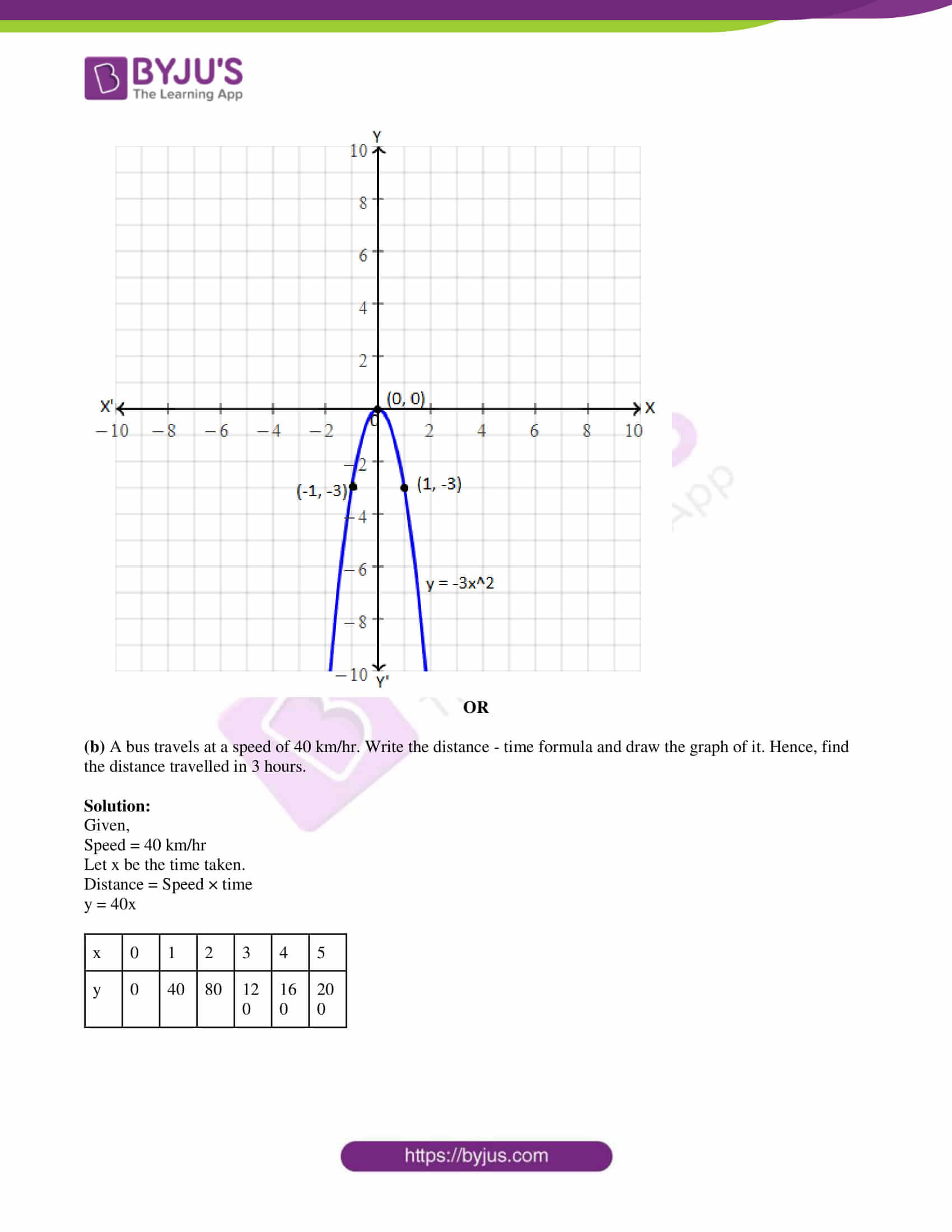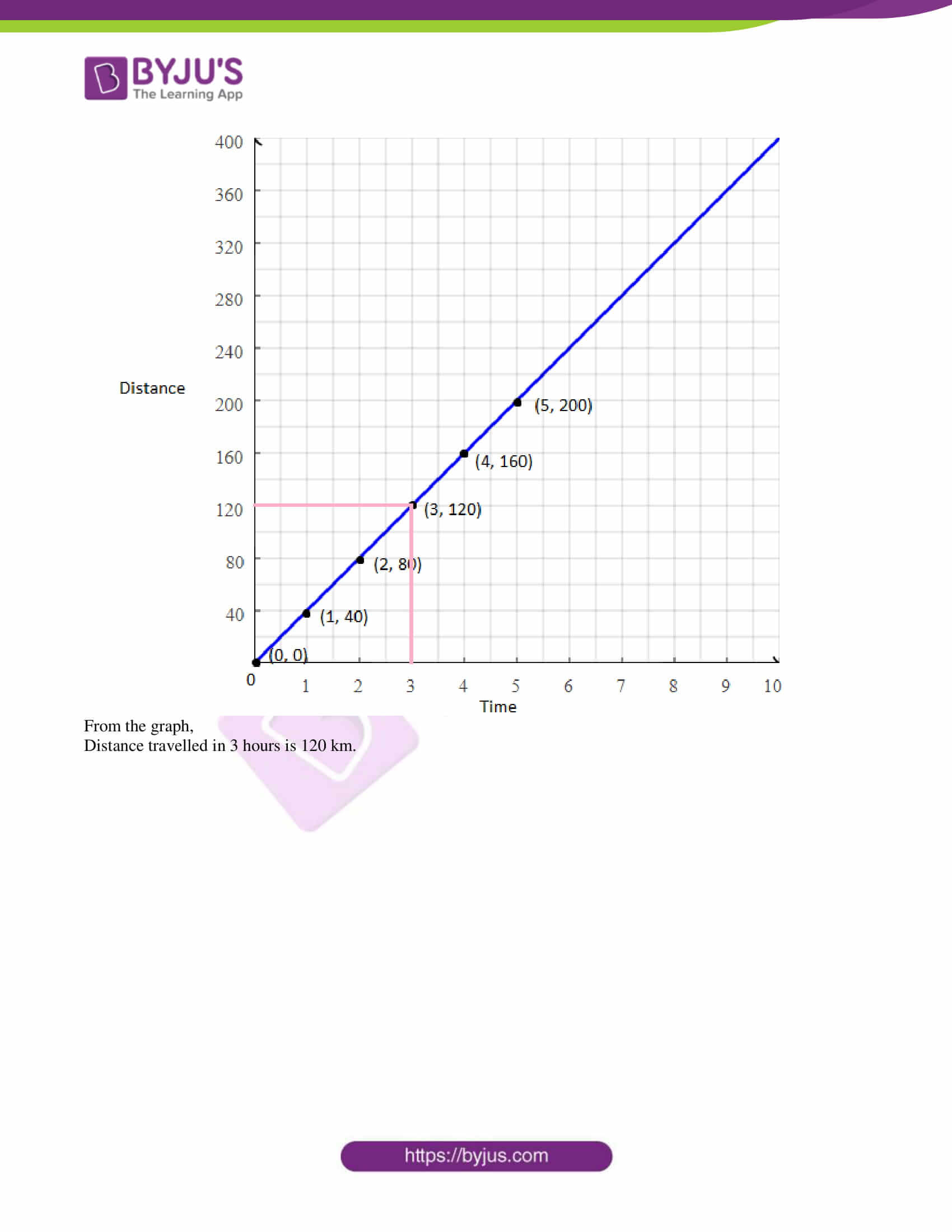SECTION – I

1. The function f: N → R is defined by f(n) = 2n. The range of the function is:

(a) the set of all even positive integers

(b) N

(c) R

(d) a subset of set of all even positive integers

Solution:

Given,

f(n) = 2n

The value of 2n is an even positive integer for all natural numbers.

Hence, the range of the function is a subset of all even possible integers.

2. If a, b, c, l, m are in AP, then the value of a – 4b + 6c – 4l + m is:

(a) 1

(b) 2

(c) 3

(d) 0

Solution:

a – 4b + 6c – 4l + m

= a + 6c + m – 4(b + l)

= a + m + 6c – 4(2c) [since b + l = 2c]

= 2c +The common ratio of the GP am – n, am, am + n is:

(a) am

(b) a-m

(c) an

(d) a-n

6c – 4(2c) [since a + m = 2c]

= 8c – 8c

= 0

3. Solution:

Given ,

am – n, am, am + n are in GP.

Common ratio = am/ am-n

= am – (m – n)

= am – m + n

= an

4. The number of polynomials having zeroes 2 and 1 is:

(a) 1

(b) 2

(c) 3

(d) more than 3

Solution:

Let p(x) = ax2 + bx + c be the quadratic polynomial.

Given, 2 and 1 are the zeroes of the polynomial.

Sum of zeroes = -b/a

2 + 1 = -b/a

⇒ -b/a = 3/1

⇒ b/a = -3/1

Thus, b = -3 and a = 1

Product of zeroes = c/a

2 × 1 = c/a

⇒ c/a = 2/1

Thus, c = 2 and a = 1

The possible expressions which satisfies the above conditions are:

p(x) = x2 – 3x + 2

p(x) = kx2 – 3kx + 2k, where k is a real number

p(x) = (x2/k) + (3/k)x + (2/k), where k is any non-zero real number

and so on.

Therefore, there exist more than 3 polynomials with zeroes 2 and 1.

5. The common root of the equations x2 – bx + c = 0 and x2 + bx – a = 0 is:

(a) (c + a)/2b

(b) (c – a)/2b

(c) (c + b)/2a

(d) (a + b)/2c

Solution:

x2 – bx + c = 0 and x2 + bx – a = 0 have a common root.

⇒ x2 – bx + c = x2 + bx – a

⇒ c + a = bx + bx

⇒ c + a = 2bx

⇒ x = (c + a)/2b

6. Which of the following statements is incorrect?

(a) A unit matrix is a scalar matrix

(b) A scalar matrix is a diagonal matrix

(c) A unit matrix is a diagonal matrix

(d) For any two matrices, the addition of matrices exists

Solution:

We know that,

The addition of two matrices exists, if they have the same order.

7. If the line segment joining the points A(3, 4) and B(14, -3) meets the x-axis at P, then the ratio in which P divides the segment AB is:

(a) 4 : 3

(b) 3 : 4

(c) 2 : 3

(d) 4 : 1

Solution:

Let P(x, 0) divide the line segment joining the points A(3, 4) and B(14, -3) in the ratio m : n.

Using section formula:

Y-coordinate of P is:

0 = [m(-3) + n(4)]/(m + n)

⇒ -3m + 4n = 0

4n = 3m

⇒ m/n = 4/3

Therefore, the required ratio is 4 : 3.

8. The distance of the point (-2, -3) from x-axis is:

(a) -2

(b) 2

(c) -3

(d) 3

Solution:

Given point is (-2, -3).

We know that the distance of any point from the x-axis is the value of y-coordinate.

Hence, the required distance is 3 units.

9. The sides of two similar triangles are in the ratio 2 : 3, then their areas are in the ratio:

(a) 9 : 4

(b) 4 : 9

(c) 2 : 3

(d) 3 : 2

Solution:

Given,

Ratio of sides of two similar triangles = 2 : 3

Ratio of their areas = Square the ratio of sides

= 22 : 32

= 4 : 9

10. The perimeters of two similar triangles are 24 cm and 18 cm respectively. If one side of the first triangle is 8 cm, then the corresponding side of the other triangle is:

(a) 4 cm

(b) 3 cm

(c) 9 cm

(d) 6 cm

Solution:

Given,

Perimeters of two similar triangles are 24 cm and 18 cm respectively.

Length of side of the first triangle = 8 cm

Let x be the side of another triangle.

Ratio of perimeters = Ratio of the corresponding sides

⇒ 24/18 = 8/x

⇒ 4/3 = 8/x

⇒ x = 24/4

⇒ x = 6

Therefore, the corresponding side of the second triangle is 6 cm.

11. 1 – [sin2θ/ (1 + cos θ)] =

(a) cos θ

(b) tan θ

(c) cot θ

(d) cosec θ

Solution:

1 – [sin2θ/ (1 + cos θ)]

= 1 – {[sin2θ)/ (1 + cos θ)] × [(1 – cos θ)/ (1 – cos θ)]}

= 1 – [sin2θ(1 – cos θ)/ (1 – cos2θ)]

= 1 – [sin2θ(1 – cos θ)/sin2θ]

= 1 – (1 – cos θ)

= 1 – 1 + cos θ

= cos θ

12. sin2θ + 1/(1 + tan2θ) =

(a) cosec2θ + cot2θ

(b) cosec2θ – cot2θ

(c) cot2θ – cosec2θ

(d) sin2θ – cos2θ

Solution:

sin2θ + 1/(1 + tan2θ)

= sin2θ + 1/sec2θ

= sin2θ + cos2θ

= 1

= cosec2θ – cot2θ [since 1 + cot2θ = cosec2θ ]

13. If the total surface area of a solid right circular cylinder is 200π cm2 and its radius is 5 cm, then the sum of its height and radius is:

(a) 20 cm

(b) 25 cm

(c) 30 cm

(d) 15 cm

Solution:

Given,

Total surface area of cylinder = 200π cm2

Radius = r = 5 cm

Let h be the height of the cylinder.

⇒ 2πr(r + h) = 200π

⇒ 5(5 + h) = 100

⇒ 5 + h = 100/5

⇒ 5 + h = 20

⇒ h = 20 – 5 = 15 cm

Sum of the radius and height = r + h = 5 + 15 = 20 cm

14. Variance of the first 11 natural numbers is:

(a) √5

(b) √10

(c) 5√2

(d) 10

Solution:

We know that variance of first n natural numbers = V = (n2 – 1)/12

VAriance for first 11 natural numbers = [(11)2 – 1]/12

= (121 – 1)/12

= 120/12

= 10

15. There are 6 defective items in a sample of 20 items. One item is drawn at random. The probability that it is a non-defective item is:

(a) 7/10

(b) 0

(c) 3/10

(d) 2/3

Solution:

Given,

Total number of items = 20

Defective items = 6

Non-defective items = 20 – 6 = 14

P(non-defective item) = 14/20 = 7/10

SECTION – II

16. Define a set.

Solution:

A set is defined as the collection of well defined objects which can be separated distinctly.

For example, S = {1, 3 , 5 , 7} is a collection of the odd numbers from 1 to 7.

17. Find the 18th and 25th terms of the sequence defined by

an = n(n + 3), in n ∈ N and n is even

an = 2n/(n2 + 1), if n ∈ N and n is odd

Solution:

18th term of the sequence:

n = 18 (even)

an = n(n + 3)

a18 = 18(18 + 3)

= 18 × 21

= 378

25th term of the sequence:

n = 25 (odd)

an = 2n/(n2 + 1)

a25 = (2 × 25)/ [(25)2 + 1]

= 50/ (625 + 1)

= 50/626

= 25/313

18. Using cross multiplication rule, solve:

3x + 5y = 25

7x + 6y = 30

Solution:

Given,

3x + 5y = 25

7x + 6y = 30

Comparing with the standard form,

a1 = 3, b1 = 5, c1 = -25

a2 = 7, b2 = 6, c2 = -30

Using cross multiplication method,

x/(b1c2 – b2c1) = y/(c1a2 – c2a1) = 1/(a1b2 – a2b1)

x/(-150 + 150) = y/(-175 + 90) = 1/(18 – 35)

x/0 = y/(-85) = 1/(-17)

x/0 = -1/17, y/(-85) = -1/17

x = 0, y = -85/-17

x = 0, y = 5

19. Form a quadratic equation whose roots are (4 + √7)/2, (4 – √7)/2.

Solution:

Let α and β be the roots of the quadratic equation.

Given, (4 + √7)/2, (4 – √7)/2 are the roots of the quadratic equation.

α + β = [(4 + √7)/2] + [(4 – √7)/2]

= [(4 + √7 + 4 – √7)/2]

= 8/2

= 4

αβ = [(4 + √7)/2] × [(4 – √7)/2]

= [(4)2 – (√7)2]/4

= (16 – 7)/4

= 9/4

Therefore, the required quadratic equation is

x2 – 4x + (9/4) = 0

⇒ 4x2 – 16x + 9 = 0

20. Define a diagonal matrix.

Solution:

Diagonal matrix is a square matrix in which every element except the principal diagonal elements is zero.

Example: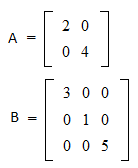21.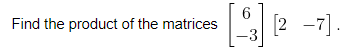Solution: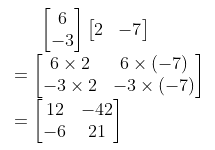22. Find the equation of the straight line passing through the points (-1, 1) and (2, -4).

Solution:

Let the given points be:

(x1, y1) = (-1, 1)

(x2, y2) = (2, -4)

Equation of the line passing through the given points is:

(y – y1)/(y2 – y1) = (x – x1)/(x2 – x1)

(y – 1)/(-4 – 1) = (x + 1)/(2 + 1)

(y – 1)/(-5) = (x + 1)/3

3(y – 1) = -5(x + 1)

3y – 3 = -5x – 5

5x + 3y – 3 + 5 = 0

5x + 3y + 2 = 0

23. Prove the identity (sin θ/cosec θ) + (cos θ/sec θ) = 1.

Solution:

LHS = (sin θ/cosec θ) + (cos θ/sec θ)

= sin θ . sin θ + cos θ . cos θ

= sin2θ + cos2θ

= 1

= RHS

Hence proved.

24. A solid right circular cylinder has radius 7 cm and height 20 cm. Find its total surface area. (take π = 22/7)

Solution:

Given,

Radius of right circular cylinder = r = 7 cm

Height = h = 20 cm

Total surface area = 2πr(r + h)

= 2 × (22/7) × 7 × (7 + 20)

= 2 × 22 × 27

= 1188 cm2

25. The volume of a cone with a circular base is 216π cm3. If the base radius is 9 cm, then find the height of the cone.

Solution:

Let h be the height of the cone.

Given,

Radius of cone = r = 9 cm

Volume = 216π cm3

(1/3)πr2h = 216π

(1/3) × 9 × 9 × h = 216

27h = 216

h = 216/27

h = 8 cm

Therefore, the height of the cone is 8 cm.

26. The standard deviation of 20 observations is √5. If each observation is multiplied by 2, find the standard deviation and variance of the resulting observations.

Solution:

Given,

Standard deviation = √5

Each observation is multiplied by 2, then new standard deviation = 2√5

Variance = (standard deviation)2

= (2√5)2

= 4 × 5

27. A die is thrown twice. Find the probability of getting a total of 9.

Solution:

Total number of outcomes = n(S) = 62 = 36

Let E be the event of getting a sum 9.

E = {(4, 5), (5, 4), (3, 6), (6, 3)}

Number of outcomes favourable to E = n(E) = 4

P(E) = n(E)/n(S)

= 4/36

= 1/9

Hence, the required probability is 1/9.

28. AB and CD are two chords of a circle which intersect each other externally at P. If AB = 4 cm, BP = 5 cm and PD = 3 cm, then find CD.

Solution:

Given,

AB and CD are two chords of a circle which intersect each other externally at P.

AB = 4 cm, BP = 5 cm and PD = 3 cm

Two chords AB and CD meet at P when produced.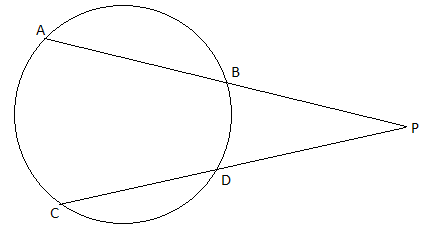⇒ PA × PB = PC × PD

⇒ (PB + AB) × PB = (PD + CD) × PD

⇒ (5 + 4) × 5 = (3 + CD) × 3

⇒ 9 × 5 = (3 + CD) × 3

⇒ (3 + CD) = 45/3

⇒ 3 + CD = 15

⇒ CD = 15 – 3

⇒ CD = 12 cm

29. A simple pendulum of length 40 cm subtends 60° at the vertex in one full oscillation. What will be the shortest distance between the initial position and the final position of the bob?

Solution:

Given,

Length of the pendulum = 40 cm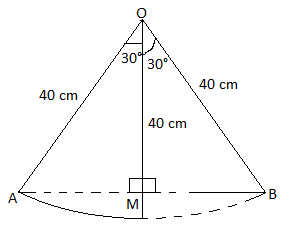In triangle OMA,

sin 30° = AM/OA

1/2 = AM/40

AM = 40/2

AM = 20 cm

AB = 2AM = 2(20) = 40 cm

Hence, the required shortest distance is 40 cm.

30. (a) Show that the function f: N → N defined by f(n) = n + 1 is not onto, by drawing arrow diagrams.

Solution:

Given,

f(n) = n + 1

f(1) = 1 + 1 = 2

f(2) = 2 + 1 = 3

f(3) = 3 + 1 = 4

f(4) = 4 + 1 = 5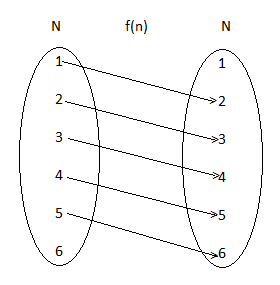Hence, the given function is not on-to.

OR

(b) Find the equation of the perpendicular to the line x = 5, passing through the point (5, 8).

Solution:

Given,

x = 5 is a vertical line.

Thus, it doesn’t have a slope.

The line perpendicular to a vertical line will be a horizontal line. Hence, its slope is 0 (zero).

Therefore, the equation of the line ia:

y – y1 = 0(x – x1) (point slope form)

y – 8 = 0(x – 5)

y – 8 = 0

y = 8

SECTION – III

31. A function f : [-7, 6) → R is defined as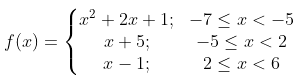Find [4f(-3) + 2f(4)]/ [f(-6) – 3f(1)].

Solution:

f(-3) = -3 + 5 = 2

f(4) = 4 – 1 = 3

f(-6) = (-6)2 + 2(-6) + 1

= 36 – 12 + 1

= 25

f(1) = 1 + 5 = 6

[4f(-3) + 2f(4)]/ [f(-6) – 3f(1)] = [4 × 2 + 2 × 3]/ [25 – 3 × 6]

= (8 + 6)/(25 – 18)

= 14/7

= 2

32. Find the sum of the series 52 + 72 + 92 + …. + 392.

Solution:

52 + 72 + 92 + …. + 392

= (12 + 22 + … + 392) – (22 + 42 + 62 + … + 380) – (12 + 32)

= (39 × 40 × 79)/6 – [4 × (19 × 20 × 39)/6] – 10

= 20540 – 9880 – 10

= 10650

33. The 4th term of a geometric sequence is 2/3 and its seventh term is 16/81. Find the geometric sequence.

Solution:

Let a be the first term and r be the common ratio of GP.

Given,

a4 = 2/3

a7 = 16/81

Now,

a7/a4 = (16/81)/ (2/3)

ar6/ar3 = (16 × 3)/ (81 × 2)

r3 = 8/27

r3 = (2/3)3

⇒ r = 2/3

Thus, ar3 = 2/3

a(2/3)3 = 2/3

a = (3/2)2

a = 9/4

Hence, the required GP is: 9/4, (9/4)(2/3), (9/4)(2/3)2,….

34. Show that the roots of the equation x2 + 2(a + b)x + 2(a2 + b2) = 0 are not real.

Solution:

Given,

x2 + 2(a + b)x + 2(a2 + b2) = 0

Δ = B2 – 4AC

= [2(a + b)]2 – 4(1)[2(a2 + b2)]

= 4(a2 + b2 + 2ab) – 8a2 – 8b2

= 4a2 + 4b2 + 8ab – 8a2 – 8b2

= -4a2 + 8ab – 4b2

= -4(a2 – 2ab + b2)

= -4(a – b)2 < 0

Therefore, the roots of the given equation are imaginary,i.e. not real.

35. The GCD of x4 + 3x3 + 5x2 + 26x + 56 and x4 + 2x3 – 4x2 – x + 28 is x2 + 5x + 7. Find their LCM.

Solution: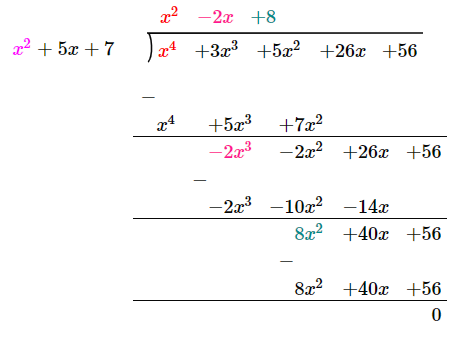Quotient = x2 – 2x + 8

LCM = (x2 – 2x + 8)(x4 + 2x3 – 4x2 – x + 28)

36. Solve: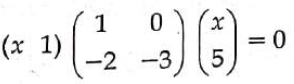Solution:

Given,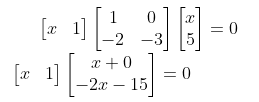(x)(x) + (1)(-2x – 15) = 0

x2 – 2x – 15 = 0

x2 – 5x + 3x – 15 = 0

x(x – 5) + 3(x – 5) = 0

(x – 5)(x + 3) = 0

x = 5, -3

37. If C is the midpoint of the line segment joining A(4, 0) and B(0, 6) and if O is the origin, then show that C is equidistant from all the vertices of ΔOAB.

Solution:

Given,

A(4, 0) and B(0, 6)

C = Midpoint of AB

= [(4 + 0)/2, (0 + 6)/2]

= (4/2, 6/2)

= (2, 3)

Let O(0, 0) be the origin.

Now,

OC = √(22 + 32) = √(4 + 9) = √13

AC = √[(2 – 4)2 + (3 – 0)2]

= √(4 + 9)

= √13

BC = √[(2 – 0)2 + (3 – 6)2]

= √(4 + 9)

= √13

Therefore, OC = AC = BC

Hence, C is equidistant from all the vertices of ΔOAB.

38. The points D and E are on the sides AB and AC of ΔABC respectively, such that DE || BC. If AB = 3AD and the area of ΔABC is 72 cm2, then find the area of the quadrilateral DBCE.

Solution:

Given,

The points D and E are on the sides AB and AC of ΔABC respectively, such that DE || BC.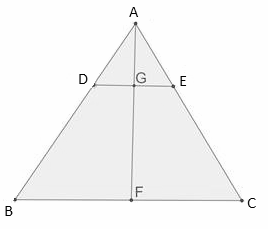∠DEA = ∠BCA (corresponding angles)

∴ ΔAED ∼ ΔACB

Similarly,

ΔAGD ∼ ΔAFB (where AF ⊥ BC)

⇒ AF = 3AG (∵ given AB = 3AD) ….(i)

Also,

BC = 3 × DE ….(ii)

Now,

(1/2) × BC × AF = (1/2) × (3 × DE) × (3 × AG) [From (i) and (ii)]

(1/2) × BC × AF = 9 × (1/2) × DE × AG

⇒ Area of triangle ABC = 9 × Area of triangle ADE

Thus, area of triangle ADE = 72/9 = 8 cm2

Area of quadrilateral BCED = Area of triangle ABC – Area of triangle ADE

= 72 – 8

= 64 cm2

39. A boy is standing at some distance from a 30 m tall building and his eye level from the ground is 1.5 m. The angle of elevation from his eye to the top of the building increases from 30° to 60° as he walks towards the building. Find the distance he walked towards the building.

Solution:

Let AB be the building.

C and D be the points of observation.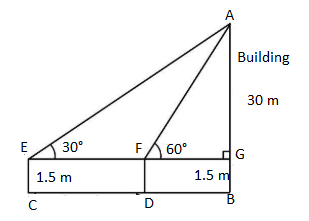AG = 30 – 1.5 = 28.5 m

In right triangle AGF,

tan 60° = AG/FG

√3 = 28.5/FG

FG = 28.5/√3

FG = 9.5√3 m

In right triangle AGE,

tan 30° = AG/GE

1/√3 = 28.5/(EF + 9.5√3)

EF + 9.5√3 = 28.5√3

EF = 28.5√3 – 9.5√3

EF = 19√3 m

Hence, the boy walked 19√3 m towards the building.

40. The diameter of a road roller of length 120 cm is 84 cm. If it takes 500 complete revolutions to level a playground, then find the cost of levelling it at the cost of 75 paise per square meter. (take π = 22/7)

Solution:

Given,

Diameter = 84 cm

Length = h = 120 cm

Radius = r = 84/2 = 42 cm

Surface area of roller = 2πrh

= 2 × (22/7) × 42 × 120

= 44 × 6 × 120

= 31680 cm2

Area covered for 500 revolutions = 31680 × 500

= 15840000 cm2

= 1584 sq.m

Cost of levelling 1 sq.m = 75 paise = Rs. 0.75

Cost of levelling the ground = 1584 × Rs. 075 = Rs. 1188

41. For a collection of data, if ∑x = 35, n = 5, ∑(x – 9)2 = 82, then find ∑x2 and ∑(x – x bar)2.

Solution:

Given,

∑x = 35, n = 5, ∑(x – 9)2 = 82

x bar = ∑x/n = 35/5 = 7

∑(x – 9)2 = 82

∑(x2 – 18x + 81) = 82

∑x2 – 18∑x + 81∑1 = 82

∑x2 – 18(35) + 81(5) = 82

∑x2 – 630 + 405 = 82

∑x2 = 630 + 82 – 405

∑x2 = 307

Similarly,

∑(x – 9)2 = 82

∑(x – 7 – 2)2 = 82

∑[(x – 7) – 2]2 = 82

∑(x – 7)2 – 4∑(x – 7) + 4∑1 = 82

∑(x – x bar)2 – 4∑(x – x bar) + 4(5) = 82

∑(x – x bar)2 – 4(0) + 20 = 82

∑(x – x bar)2 = 82 – 20

∑(x – x bar)2 = 62

42. The probability that A, B and C can solve a problem are 4/5, 2/3 and 3/7 respectively. The probability of the problem being solved by A and B is 8/15, B and C is 2/7, A and C is 12/35. The probability of the problem being solved by all the three is 8/35. Find the probability that the problem can be solved by at least one of them.

Solution:

Given,

P(A) = 4/5

P(B) = 2/3

P(C) = 3/7

P(A ⋂ B) = 8/15

P(B ⋂ C) = 2/7

P(A ⋂ C) = 12/35

P(A ⋂ B ⋂ C) = 8/35

P(A ⋃ B ⋃ C) = P(A) + P(B) + P(C) – P(A ⋂ B) – P(B ⋂ C) – P(A ⋂ C) + P(A ⋂ B ⋂ C)

= (⅘) + (⅔) + (3/7) – (8/15) – (2/7) – (12/35) + (8/35)

= (84 + 70 + 45 – 56 – 30 – 36 + 24)/105

= 101/105

Hence, the probability of the problem can be solved by at least one of them is 101/105.

43. A triangle has vertices at (6, 7), (2, -9) and (-4, 1). Find the slopes of its medians.

Solution:

Let A(6, 7), B(2, -9) and C(-4, 1) be the vertices of triangle ABC.

BD, CE and AF be the medians of AC, AB and BC respectively.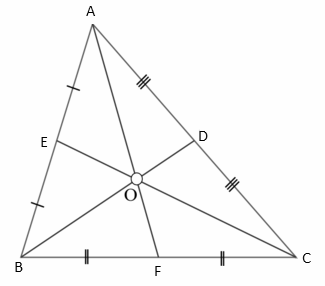D = Midpoint of AC

= [(6 – 4)/2, (7 + 1)/2]

= (2/2, 8/2)

= (1, 4)

E = Midpoint of AB

= [(6 + 2)/2, (7 – 9)/2]

= (8/2, -2/2)

= (4, -1)

F = Midpoint of BC

= [(2 – 4)/2, (-9 + 1)/2]

= (-2/2, -8/2)

= (-1, -4)

Slope of median AF = (-4 – 7)/(-1 – 6) = 11/7

Slope of median BD = (4 + 9)/ (1 – 2) = -13

Slope of median CE = (-1 – 1)/ (4 + 4) = -2/8 = -¼

44. A hollow cylinder pipe is of length 40 cm. Its internal and external radii are 4 cm and 12 cm respectively. It is melted and cast into a solid cylinder of length 20 cm. Find the radius of the new cylinder.

Solution:

Given,

Length of hollow cylinder = H = 40 cm

Internal radius = r = 4 cm

External radius = R = 12 cm

Length of the solid cylinder = h = 20 cm

Let r2 be the radius of the solid cylinder.

Volume of solid cylinder = Volume of hollow cylinder

πr22h = πh(R2 – r2)

r22 × 20 = 40[(12)2 – (4)2]

r22 = 2[144 – 16]

r22 = 2 × 128

r22 = 256

r2 = 16 cm

Hence, the required radius is 16 cm.

45. (a) Prove that A\(B ⋃ C) = (A\B) ⋂ (A\C) by using Venn diagrams.

Solution: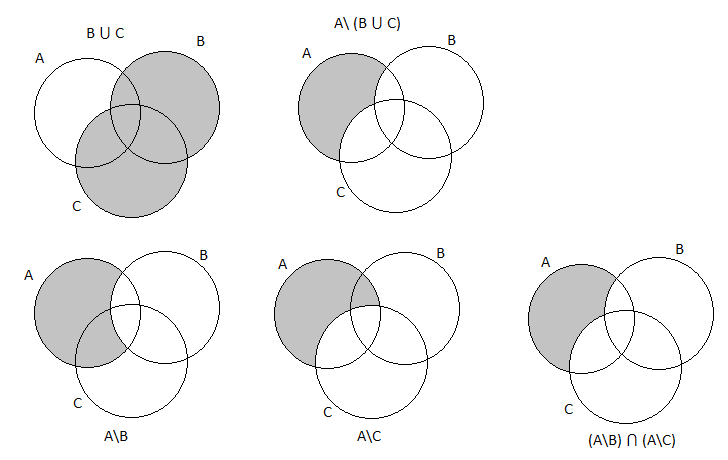Hence, proved that: A\(B ⋃ C) = (A\B) ⋂ (A\C)

OR

(b) If the remainder on division x3 + 2x2 + kx + 3 by x – 3 is 21, find the value of k and hence find the zeroes of the cubic polynomial x3 + 2x2 + kx – 18.

Solution:

Given,

p(x) = x3 + 2x2 + kx + 3

g(x) = x – 3

r(x) = 21

Thus, p(3) = 21

(3)3 + 2(3)2 + k(3) + 3 = 21

27 + 18 + 3k + 3 = 21

48 + 3k = 21

3k = 21 – 48

k = -27/3

k = -9

Therefore, the cubic polynomial is f(x) = x3 + 2x2 + (-9)x – 18 = x3 + 2x2 – 9x – 18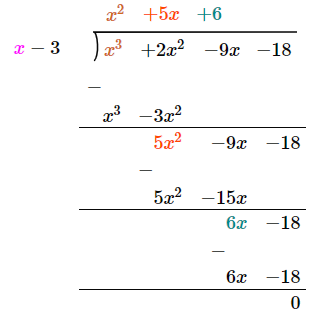Consider,

x2 + 5x + 6 = 0

x2 + 2x + 3x + 6 = 0

x(x + 2) + 3(x + 2) = 0

(x + 2)(x + 3) = 0

x = -2, x = -3

Hence, the required zeroes of the cubic polynomial are 3, -2, -3.

SECTION – IV

46. (a) Draw a circle of radius 4.8 cm. Take a point on the circle, draw the tangent at that point using the tangent-chord theorem.

Solution: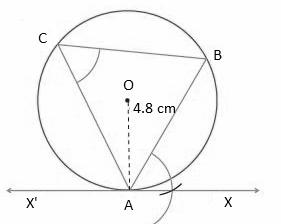Hence, XAX’ is the required tangent at A on the circle of radius 4.8 cm.

OR

(b) Construct a cyclic quadrilateral ABCD in which AB = 6 cm, AC = 7 cm, BC = 6 cm and AD = 4.2 cm.

Solution: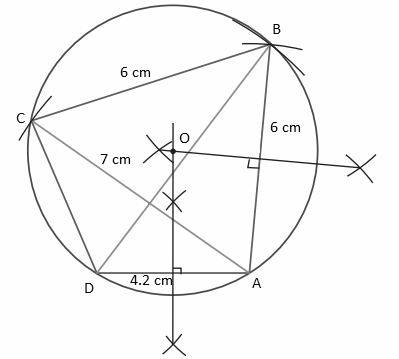47. (a) Draw the graph of y = -3x2.

Solution:

Given,

y = -3x2

Table for the corresponding x and y values:

 x -1 0 1 y -3 0 -3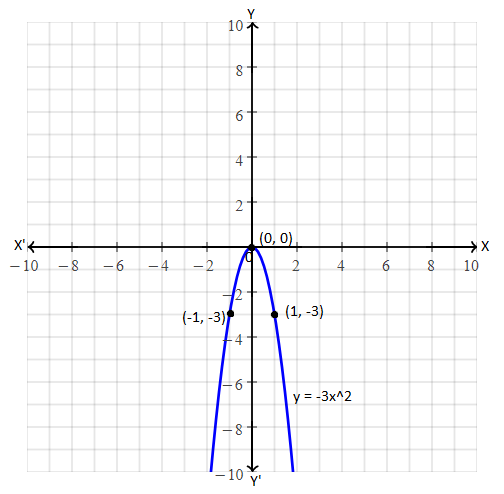OR

(b) A bus travels at a speed of 40 km/hr. Write the distance – time formula and draw the graph of it. Hence, find the distance travelled in 3 hours.

Solution:

Given,

Speed = 40 km/hr

Let x be the time taken.

Distance = Speed × time

y = 40x

 x 0 1 2 3 4 5 y 0 40 80 120 160 200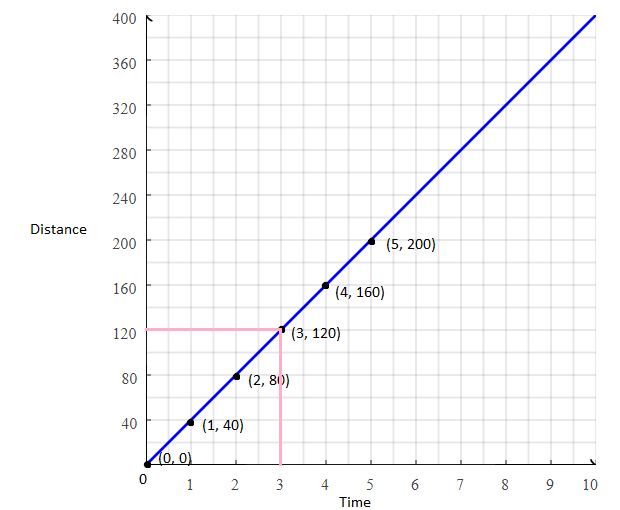From the graph,

Distance travelled in 3 hours is 120 km.